Predict house prices - deep learning, keras

Overview

In this notebook I fool around with some fast prototype models for predicting house prices using deep neural nets!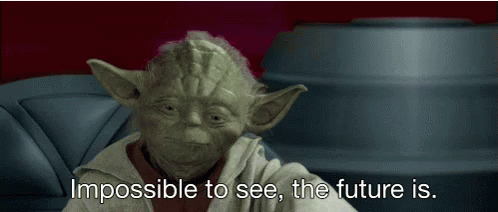First I will do some LSTM modelling for the house price movement using tidy backtested crossvalidation.

Next I use tidy crossvalidated dense feed forward networks to predict the price of a property based on the properties descriptions!

Coming soon; I will use the LSTM model to produce a 1 month forecast and feed this as a dimension to the inference model during predictions of houses for which we believe we have the descriptive data now but we will only sell the property in a months time. I will compare the errors by holding out the last few months of data to test the combined accuracy accounting for price movement.

Naive model (no time index)

As a little introduction we can cover the example in the book by François Chollet with J. J. Allaire

For our first model we will be using the boston housing data from 1970. This data collected features of the sale but had no time index - probably because the data was only collected over 1970; possibly with some cencoring.

With no time index in the data we can think of the tensors as being 1D tensors since the samples have n features on only 1 axis.

With this model we cannot really predict into the future so our predictions are only valid for predicting house prices right now (1970).

dataset <- dataset_boston_housing()
c(c(train_data, train_targets), c(test_data, test_targets)) %<-% dataset

The training data we load here is already in a matrix format for us:

##         [,1] [,2]  [,3] [,4]  [,5]  [,6]  [,7]   [,8] [,9] [,10] [,11]
## [1,] 1.23247  0.0  8.14    0 0.538 6.142  91.7 3.9769    4   307  21.0
## [2,] 0.02177 82.5  2.03    0 0.415 7.610  15.7 6.2700    2   348  14.7
## [3,] 4.89822  0.0 18.10    0 0.631 4.970 100.0 1.3325   24   666  20.2
## [4,] 0.03961  0.0  5.19    0 0.515 6.037  34.5 5.9853    5   224  20.2
## [5,] 3.69311  0.0 18.10    0 0.713 6.376  88.4 2.5671   24   666  20.2
## [6,] 0.28392  0.0  7.38    0 0.493 5.708  74.3 4.7211    5   287  19.6
##       [,12] [,13]
## [1,] 396.90 18.72
## [2,] 395.38  3.11
## [3,] 375.52  3.26
## [4,] 396.90  8.01
## [5,] 391.43 14.65
## [6,] 391.13 11.74

We have 13 features/dimensions of collected data for each sample that we can use to predict the house price:

##  15.2 42.3 50.0 21.1 17.7 18.5

Scale the variables

mean <- apply(train_data, 2, mean)
std <- apply(train_data, 2, sd)
train_data <- scale(train_data, center = mean, scale = std)
test_data <- scale(test_data, center = mean, scale = std)

Define the model

We can start by defining the model. Since we will be using cross-validation we define a function that constructs the model. We do this because we need to construct and train a model on each fold so we can gauge the performance accross the different folds as we fine tune our model:

Function that constructs a model

Note that I am assuming the data input is a resample object… That’s because I will be creating a tidy crossvalidation and so the objects in my table will be resamples…

I am also assuming the outcome is called ‘target’, but this can be generalised using enquo

define_compile_train_predict <- function(train,test,epochs = 100,shuffle = TRUE,...){

train_X <- train %>% as_tibble() %>% select(-target) %>% as.matrix()
test_X <- test %>% as_tibble() %>% select(-target) %>% as.matrix()
train_y <- train %>% as_tibble() %>%  select(target) %>% as.matrix()
test_y <- test %>% as_tibble() %>%  select(target) %>% as.matrix()

#define
base_model <-
keras_model_sequential() %>%
layer_dense(units = 64, activation = 'relu', input_shape = c(13)) %>% # 1 axis and nr features = number columns
layer_batch_normalization() %>%
# layer_dropout(rate = 0.2) %>%
layer_dense(units = 64, activation ='relu') %>%
# layer_dense(units = 25, activation ='relu') %>%
layer_dense(units = 1)

#compile
base_model %>% compile(
loss='mse',
optimizer='rmsprop',
metrics = c('mae')
)

#train
history <- base_model %>%
keras::fit(train_X,
train_y,
epochs=epochs,
shuffle=shuffle,
validation_data= list(test_X, test_y),
verbose = 0
)

#predict
predictions <-
base_model %>%
keras::predict_on_batch(x = train_X)

results <-
base_model %>%
keras::evaluate(test_X, test_y, verbose = 0)

plot_outp <-
history %>% plot

return(list(predictions = predictions, loss = results$loss, mae = results$mean_absolute_error, plot_outp = plot_outp,base_model=base_model,history = history))

}

In the example in the book they use a for loop to set up the different folds and then apply the training and validation inside these loops. Since R has a lot of this already solved in tidy packages I am just going to leverage them and stay inside of a tidy format so we can keep all the intermediate results and visualize the outputs all in one go

Construct tidy cross-validation

To do this we first setup a nice tibble using the modelr package:

tidy_cross_val <-
train_data %>%
as_tibble() %>%
bind_cols(target = train_targets) %>%
modelr::crossv_kfold(k = 5) %>%
rename(fold = .id) %>%
select(fold,everything())

tidy_cross_val
## # A tibble: 5 x 3
##   fold  train          test
##   <chr> <list>         <list>
## 1 1     <S3: resample> <S3: resample>
## 2 2     <S3: resample> <S3: resample>
## 3 3     <S3: resample> <S3: resample>
## 4 4     <S3: resample> <S3: resample>
## 5 5     <S3: resample> <S3: resample>

Now we can build the models and also compile them, after that we just pluck out the stuff we stored in our function:

# tidy_cross_val$model_output %>% map_dbl(pluck,"mae") tidy_cross_val <- tidy_cross_val %>% mutate(model_output = pmap(list(train = train,test = test), define_compile_train_predict,epochs = 200)) %>% mutate(mae = model_output %>% map_dbl(pluck,"mae")) %>% mutate(plot_outp = model_output %>% map(pluck,"plot_outp")) %>% mutate(loss = model_output %>% map_dbl(pluck,"loss")) %>% mutate(predictions = model_output %>% map(pluck,"predictions")) %>% select(everything(),model_output) tidy_cross_val ## # A tibble: 5 x 8 ## fold train test model_output mae plot_outp loss predictions ## <chr> <list> <list> <list> <dbl> <list> <dbl> <list> ## 1 1 <S3: res~ <S3: re~ <list > 2.41 <S3: gg> 10.5 <dbl [323 x~ ## 2 2 <S3: res~ <S3: re~ <list > 2.17 <S3: gg> 12.6 <dbl [323 x~ ## 3 3 <S3: res~ <S3: re~ <list > 2.24 <S3: gg> 8.56 <dbl [323 x~ ## 4 4 <S3: res~ <S3: re~ <list > 2.74 <S3: gg> 13.9 <dbl [323 x~ ## 5 5 <S3: res~ <S3: re~ <list > 2.55 <S3: gg> 10.9 <dbl [324 x~ By mapping the deeplearning model over all these folds of data we store our results directly into this table. That means we can do interesting things like plot them altogether: tidy_cross_val %>% ggplot(aes(x=fold,y=mae, fill = fold))+ geom_bar(stat="identity")+ ggtitle("The k-fold accuracy of the deep neural net",subtitle = "Not yet scaled back...")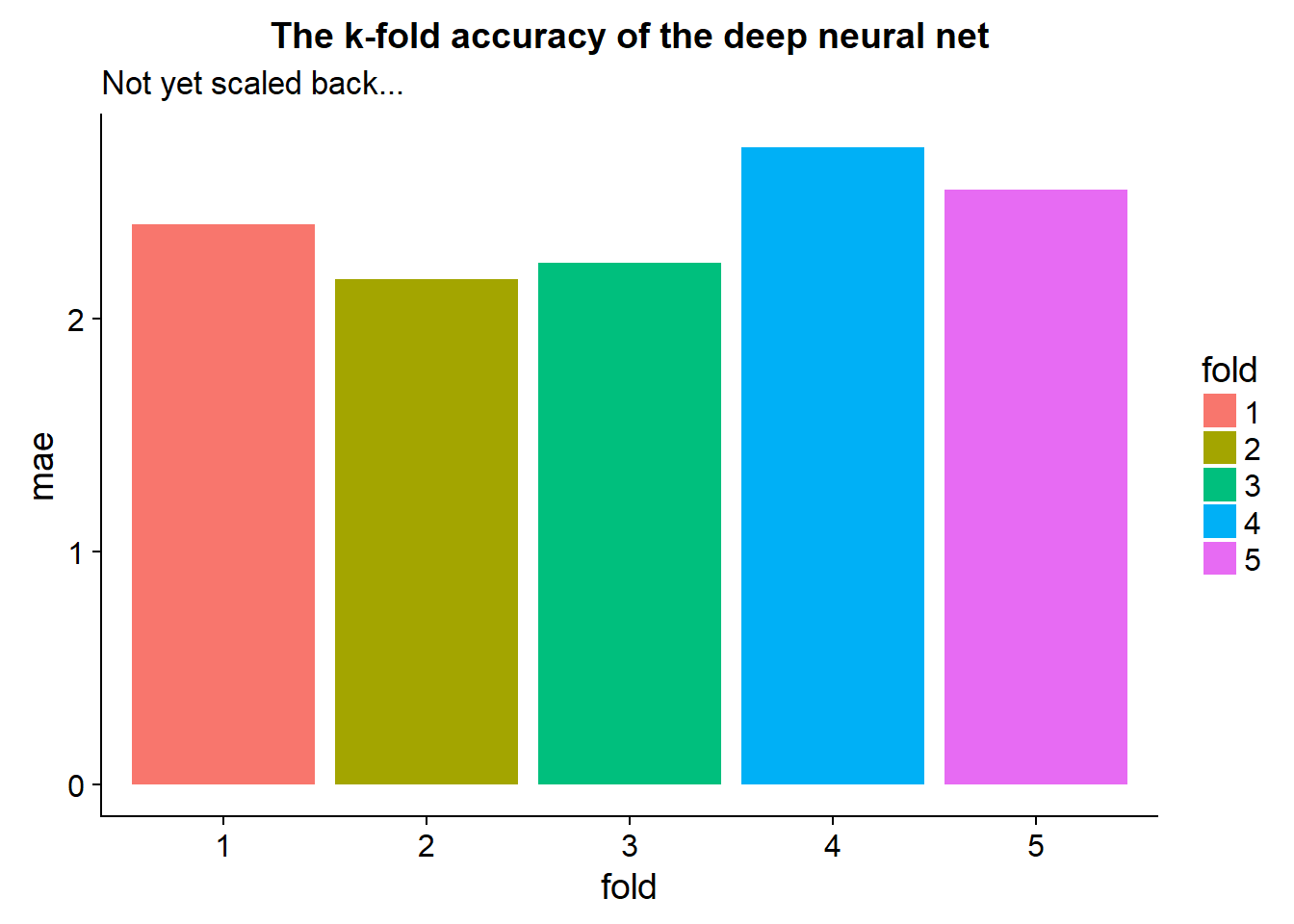This gives us a good idea of our certainty in the model. From our tidy cross-validation output we may notice some extreme values for folds in terms of high mae or loss… These should be treated appropriately Let’s take a closer look at the max mae fold: tidy_cross_val %>% filter(.$mae == max(.$mae)) %>% pull(plot_outp) ## []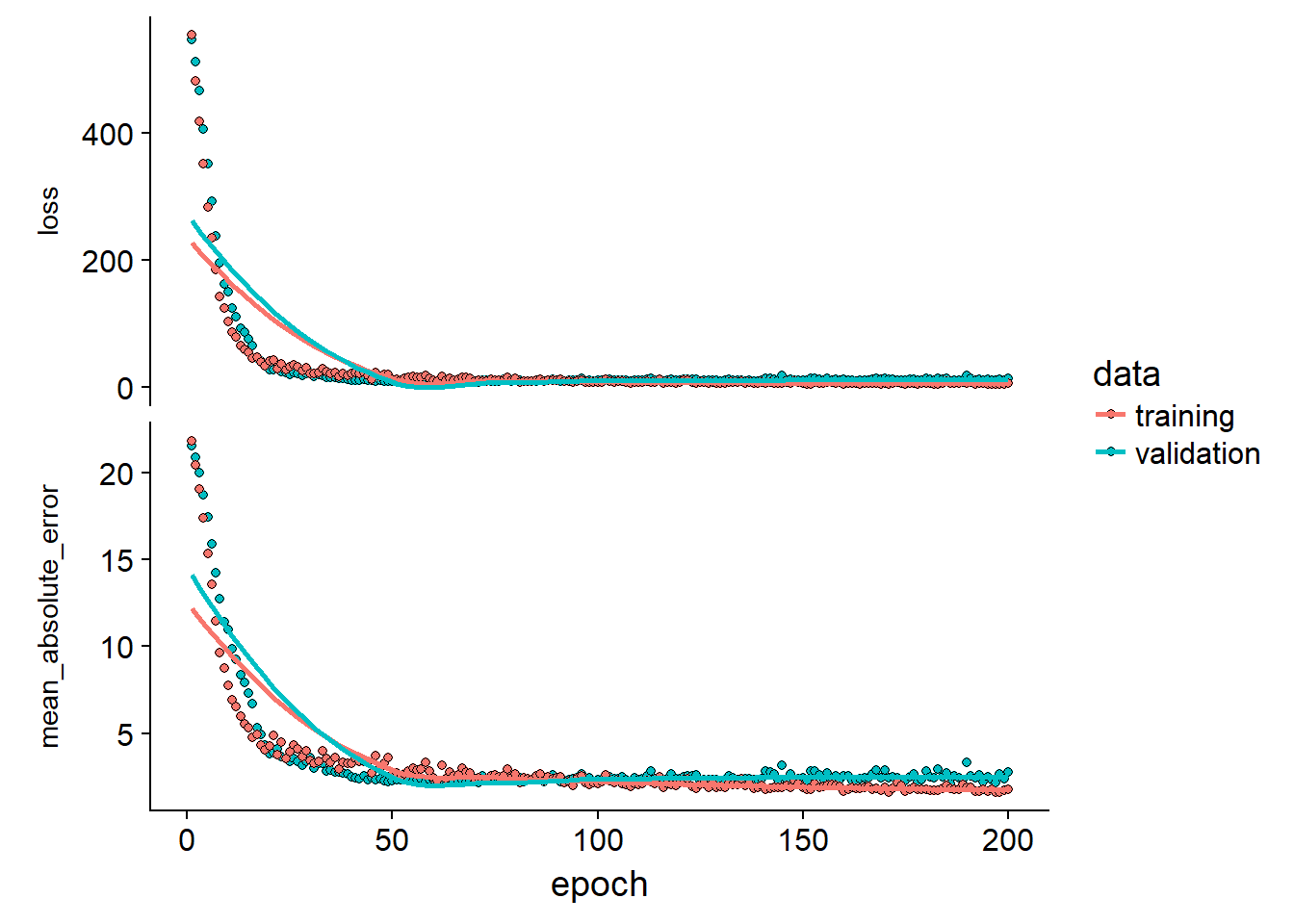We can look at some extreme folds to see if the validation and training loss diverges which should indicate over-fitting. In this case the model seems to fit great Measuring over-fit using k-folds crossvalidation We can visualize this over-fitting more generally by looking in the history object we saved from the keras::fit function. By taking the mae at each epoch for all the folds we can get the average mae over the folds at each epoch and visualize how long we should train before we start making some spurious predictions: all_mae_histories <- tidy_cross_val$model_output %>% map_df(pluck,"history","metrics","val_mean_absolute_error") %>% t()

average_mae_history <- data.frame(
epoch = seq(1:ncol(all_mae_histories)),
validation_mae = apply(all_mae_histories, 2, mean)
)

average_mae_history %>%
ggplot(aes(x = epoch, y = validation_mae, color = epoch))+
geom_line()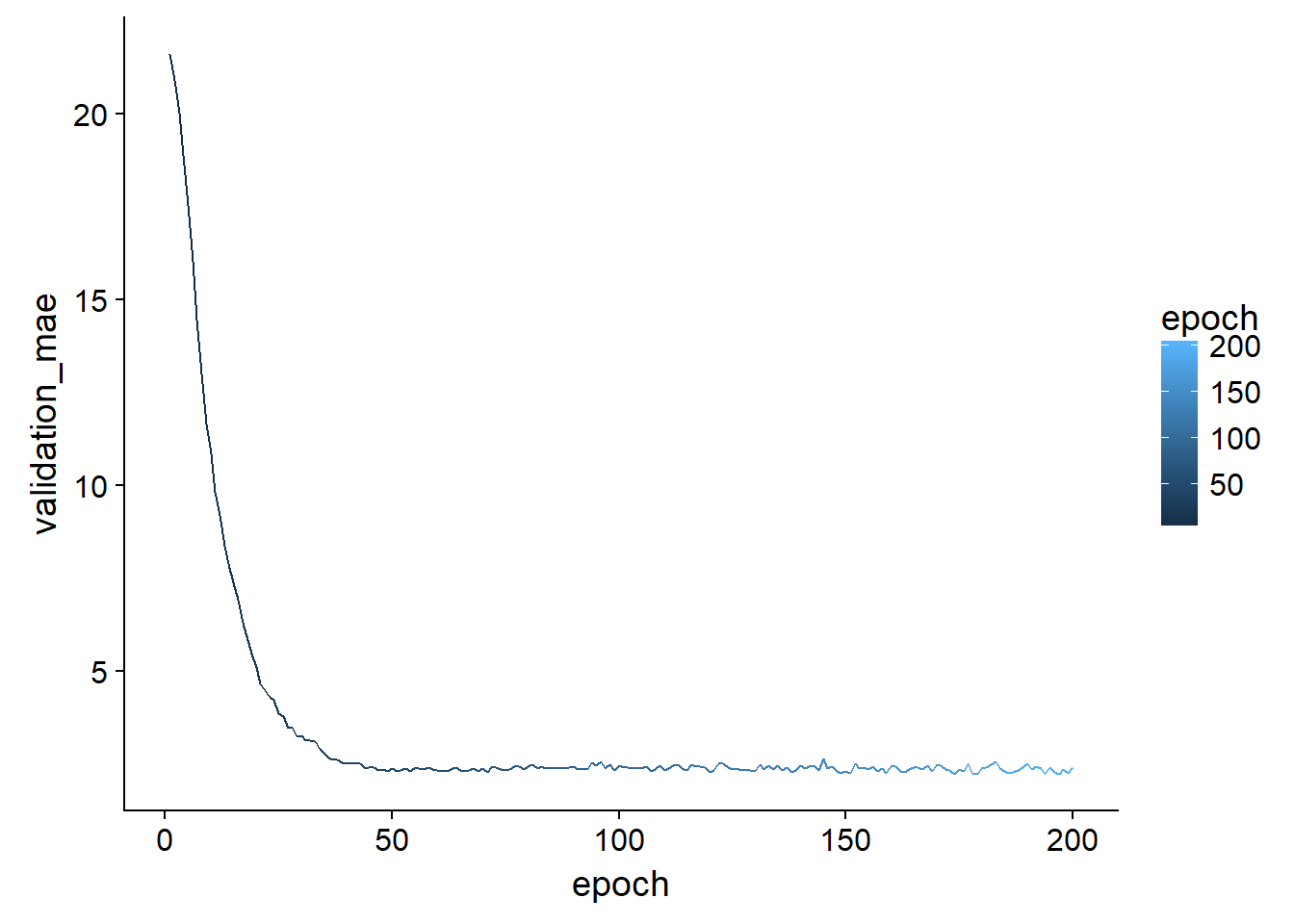average_mae_history %>%
ggplot(aes(x = epoch, y = validation_mae, color = epoch))+
geom_smooth()
## geom_smooth() using method = 'loess'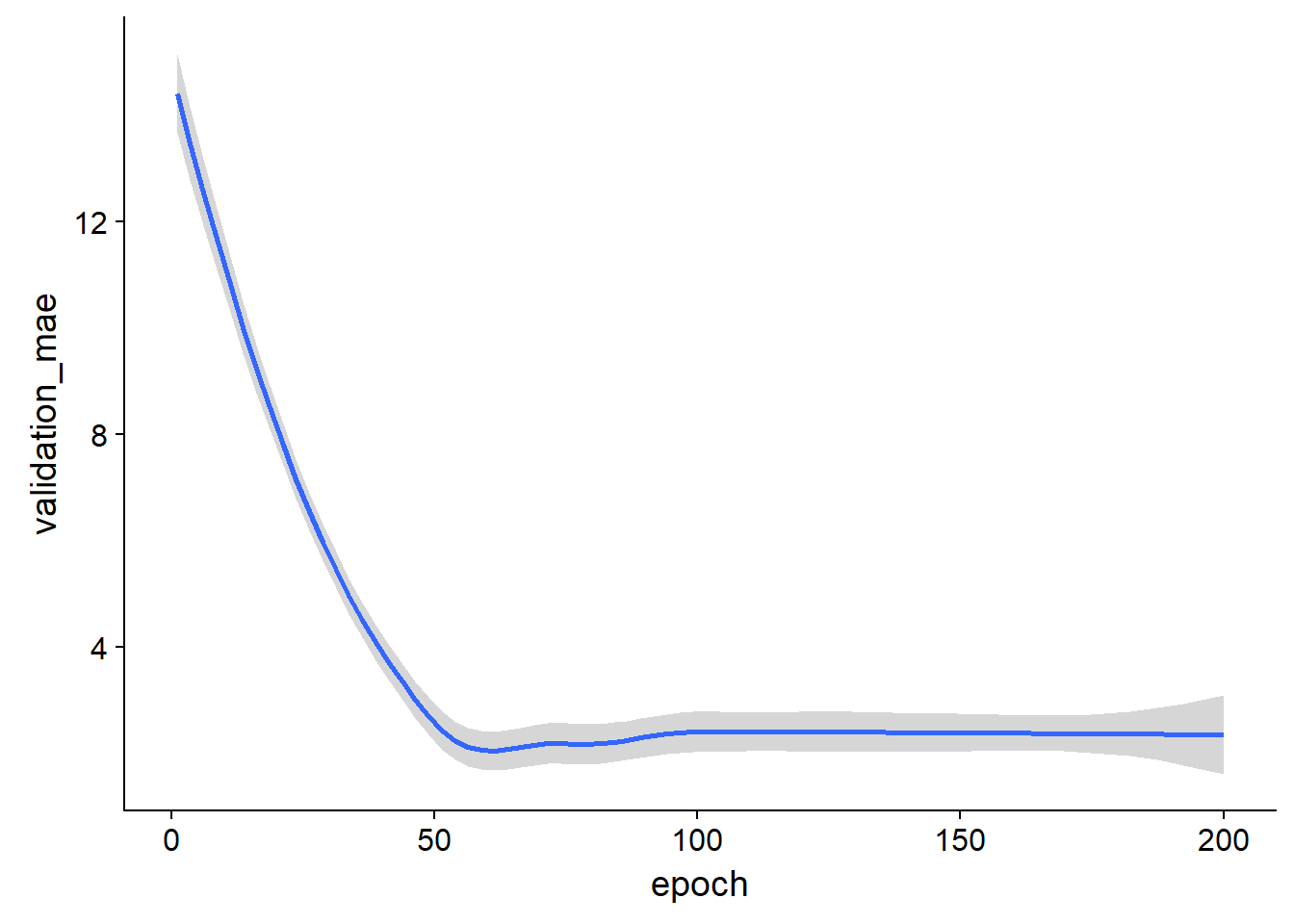The benefit of this is that you are plotting the mean behaviour of the training at each epoch over all folds

Get results

Once we are happy with our model we can pull out all the results like this:

tidy_cross_val %>%
select(train,predictions) %>%
mutate(train = train %>% map(as_tibble)) %>%
unnest(.drop = TRUE) %>%
## # A tibble: 6 x 15
##   predictions       V1     V2     V3     V4     V5     V6     V7     V8
##         <dbl>    <dbl>  <dbl>  <dbl>  <dbl>  <dbl>  <dbl>  <dbl>  <dbl>
## 1        16.7 -0.272   -0.483 -0.435 -0.257 -0.165 -0.176  0.812  0.117
## 2        54.0  0.125   -0.483  1.03  -0.257  0.628 -1.83   1.11  -1.19
## 3        21.4 -0.401   -0.483 -0.868 -0.257 -0.361 -0.324 -1.24   1.11
## 4        18.6 -0.00563 -0.483  1.03  -0.257  1.33   0.153  0.694 -0.578
## 5        18.8 -0.375   -0.483 -0.547 -0.257 -0.549 -0.788  0.189  0.483
## 6        10.8  0.589   -0.483  1.03  -0.257  1.22  -1.03   1.11  -1.06
## # ... with 6 more variables: V9 <dbl>, V10 <dbl>, V11 <dbl>, V12 <dbl>,
## #   V13 <dbl>, target <dbl>

But we have only predicted on the training data in our function and we used the model trained only on that fold… Instead it would make a lot more sense to train the model on all the data now that we have tested and tuned the model (possibly censor bad folds?)

Using all the data

Run model:

train <-
train_data %>%
as_tibble() %>%
bind_cols(target = train_targets)

test <-
test_data %>%
as_tibble() %>%
bind_cols(target = test_targets)

results <-
define_compile_train_predict(train,test,epochs = 100)

print('mae:')
##  "mae:"
results$mae ##  2.429138 print('loss:') ##  "loss:" results$loss
##  14.56804
results$plot_outp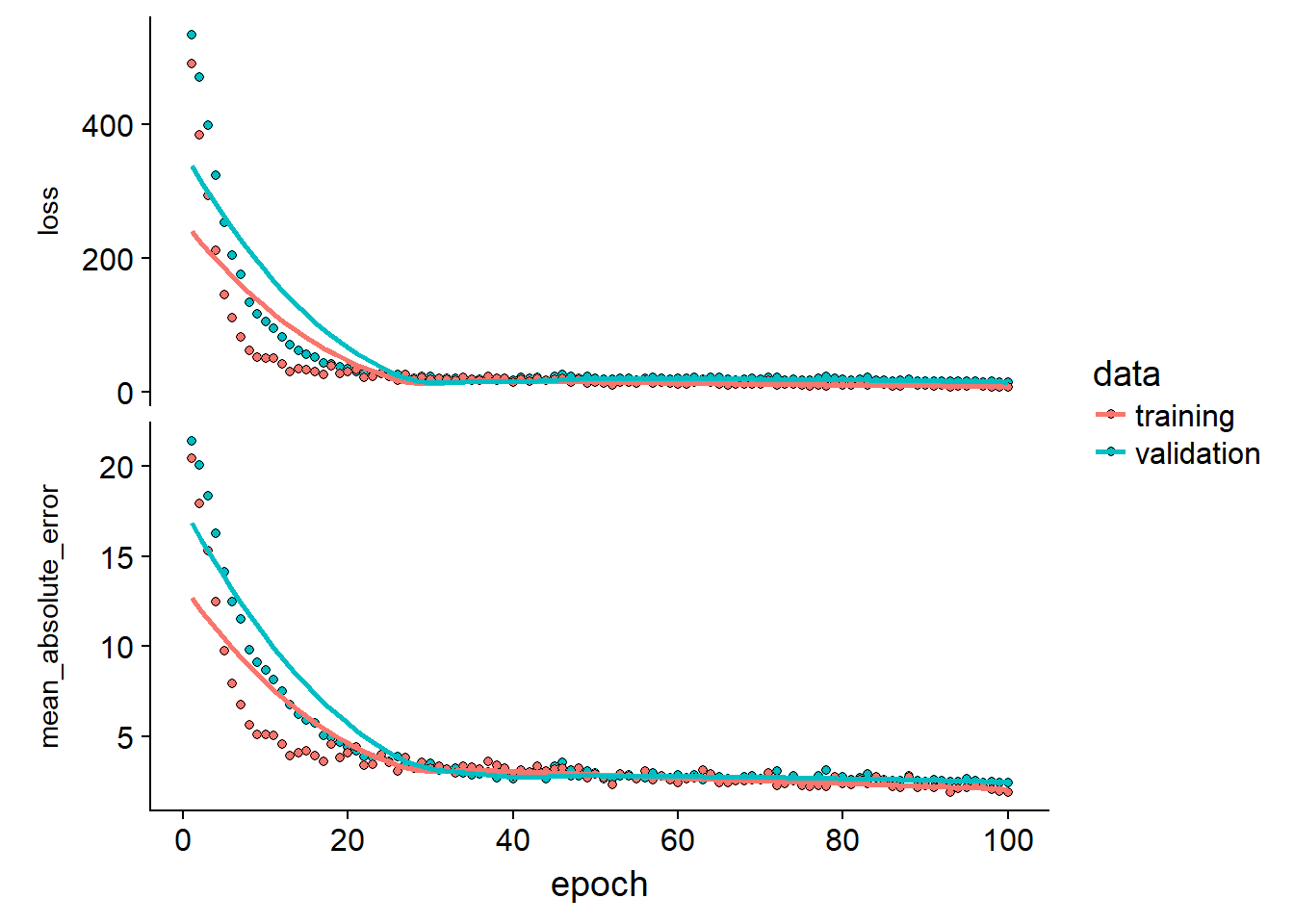Get predictions: output_data <- bind_cols(predictions = results$predictions,train["target"],train)

## # A tibble: 6 x 16
##   predictions target       V1     V2     V3     V4     V5     V6     V7
##         <dbl>  <dbl>    <dbl>  <dbl>  <dbl>  <dbl>  <dbl>  <dbl>  <dbl>
## 1        17.3   15.2 -0.272   -0.483 -0.435 -0.257 -0.165 -0.176  0.812
## 2        43.4   42.3 -0.403    2.99  -1.33  -0.257 -1.21   1.89  -1.91
## 3        51.4   50.0  0.125   -0.483  1.03  -0.257  0.628 -1.83   1.11
## 4        22.3   21.1 -0.401   -0.483 -0.868 -0.257 -0.361 -0.324 -1.24
## 5        18.4   17.7 -0.00563 -0.483  1.03  -0.257  1.33   0.153  0.694
## 6        20.8   18.5 -0.375   -0.483 -0.547 -0.257 -0.549 -0.788  0.189
## # ... with 7 more variables: V8 <dbl>, V9 <dbl>, V10 <dbl>, V11 <dbl>,
## #   V12 <dbl>, V13 <dbl>, target1 <dbl>

Let’s visualize the accuracy of this model:

output_data %>%
arrange(target) %>%
mutate(perfect = seq(5,50,length.out = nrow(.))) %>%
mutate(estimate = ifelse(target > predictions,"underestimated","overestimated")) %>%
select(predictions,target,estimate,perfect) %>%
ggplot()+
geom_point(aes(x = predictions, y = target, color = estimate))+
geom_line(aes(x=perfect, y = perfect))+
ggtitle("Model accuracy")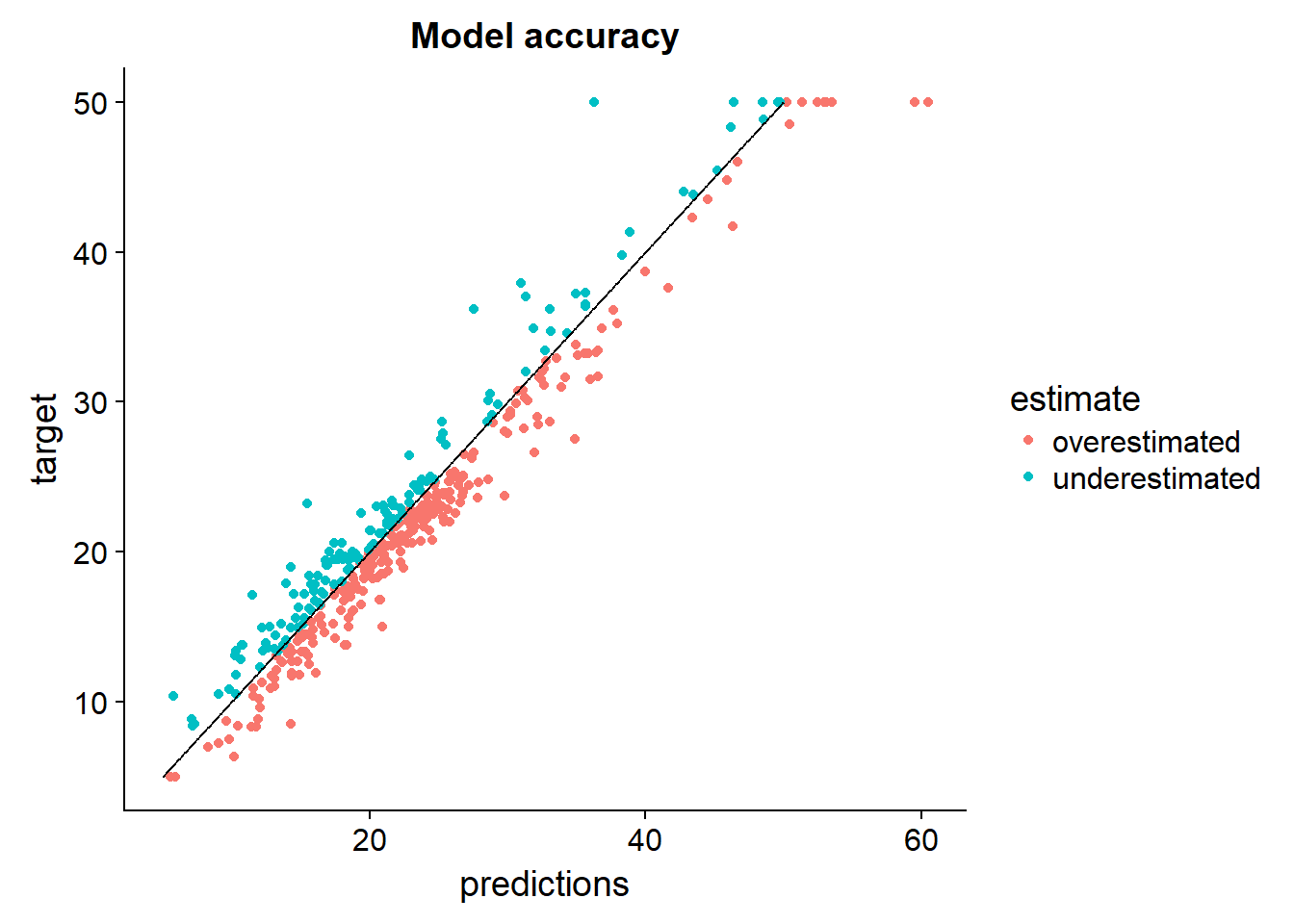What can we say about the bias of the model:

output_data %>%
arrange(target) %>%
mutate(perfect = seq(5,50,length.out = nrow(.))) %>%
mutate(estimate = ifelse(target > predictions,"underestimated","overestimated")) %>%
select(predictions,target,estimate,perfect) %>%
group_by(estimate) %>%
tally %>%
ggplot()+
geom_bar(aes(x= estimate, y=n, fill = estimate),stat="identity")+
ggtitle("Low model error but upward bias")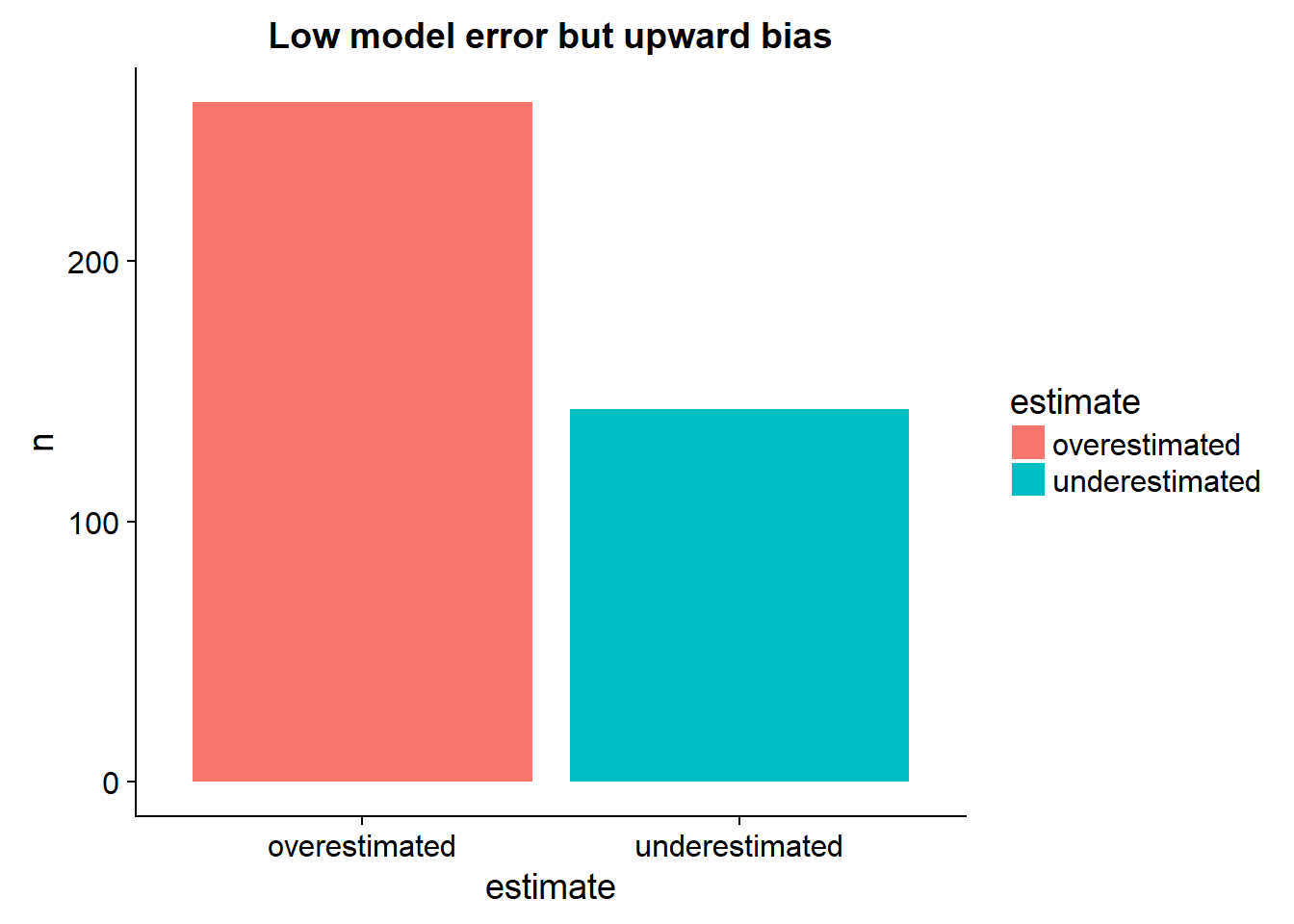train <-
train_data %>%
as_tibble() %>%
bind_cols(target = train_targets %>% as.numeric() )

test <-
test_data %>%
as_tibble() %>%
bind_cols(target = test_targets %>% as.numeric() )

tr_contrl <- caret::trainControl(method = 'cv', number = 5)

results <-
caret::train(form = target~., data = train,method = 'gbm',trControl = tr_contrl)
results %>% summary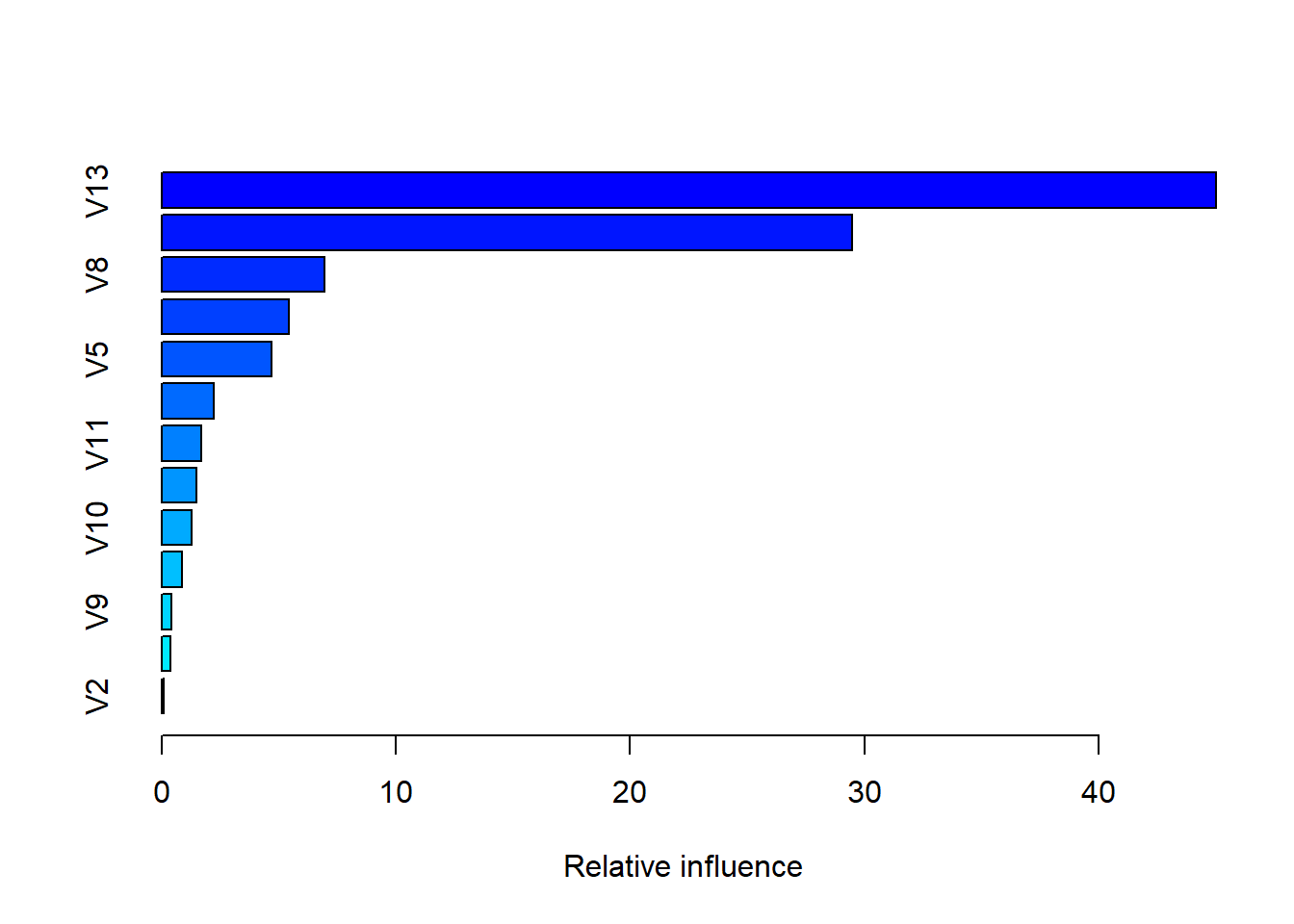##     var     rel.inf
## V13 V13 45.00698622
## V6   V6 29.45790750
## V8   V8  6.97044377
## V1   V1  5.42153107
## V5   V5  4.70147030
## V7   V7  2.23913360
## V11 V11  1.66965671
## V12 V12  1.46723050
## V10 V10  1.28689723
## V4   V4  0.87476817
## V9   V9  0.42687149
## V3   V3  0.37745176
## V2   V2  0.09965169
results
##
## 404 samples
##  13 predictor
##
## No pre-processing
## Resampling: Cross-Validated (5 fold)
## Summary of sample sizes: 323, 323, 323, 323, 324
## Resampling results across tuning parameters:
##
##   interaction.depth  n.trees  RMSE      Rsquared   MAE
##   1                   50      4.397289  0.7634246  2.947976
##   1                  100      3.964773  0.8032464  2.700262
##   1                  150      3.862273  0.8106288  2.670015
##   2                   50      3.868630  0.8136770  2.621053
##   2                  100      3.638292  0.8332715  2.537585
##   2                  150      3.562024  0.8394754  2.497162
##   3                   50      3.611696  0.8389383  2.463967
##   3                  100      3.350380  0.8589171  2.345241
##   3                  150      3.214416  0.8688836  2.286692
##
## Tuning parameter 'shrinkage' was held constant at a value of 0.1
##
## Tuning parameter 'n.minobsinnode' was held constant at a value of 10
## RMSE was used to select the optimal model using the smallest value.
## The final values used for the model were n.trees = 150,
##  interaction.depth = 3, shrinkage = 0.1 and n.minobsinnode = 10.

So the gradient boosted machine using k-fold can get around 2.4-2.9 MAE and the GBM model is historically the best tabular learning paradigm in the average kaggle contest the last couple of years (around 2016). Our model learned the same ammount of accuracy also using cross-validation.Let’s visualize the accuracy of this model:

output_data <-
train %>%
as_tibble() %>%
mutate(predictions = results %>% predict(newData=train %>% select(-target))) %>%
select(target,predictions)

output_data %>%
arrange(target) %>%
mutate(perfect = seq(5,50,length.out = nrow(.))) %>%
mutate(estimate = ifelse(target > predictions,"underestimated","overestimated")) %>%
select(predictions,target,estimate,perfect) %>%
ggplot()+
geom_point(aes(x = predictions, y = target, color = estimate))+
geom_line(aes(x=perfect, y = perfect))+
ggtitle("Model accuracy GBM")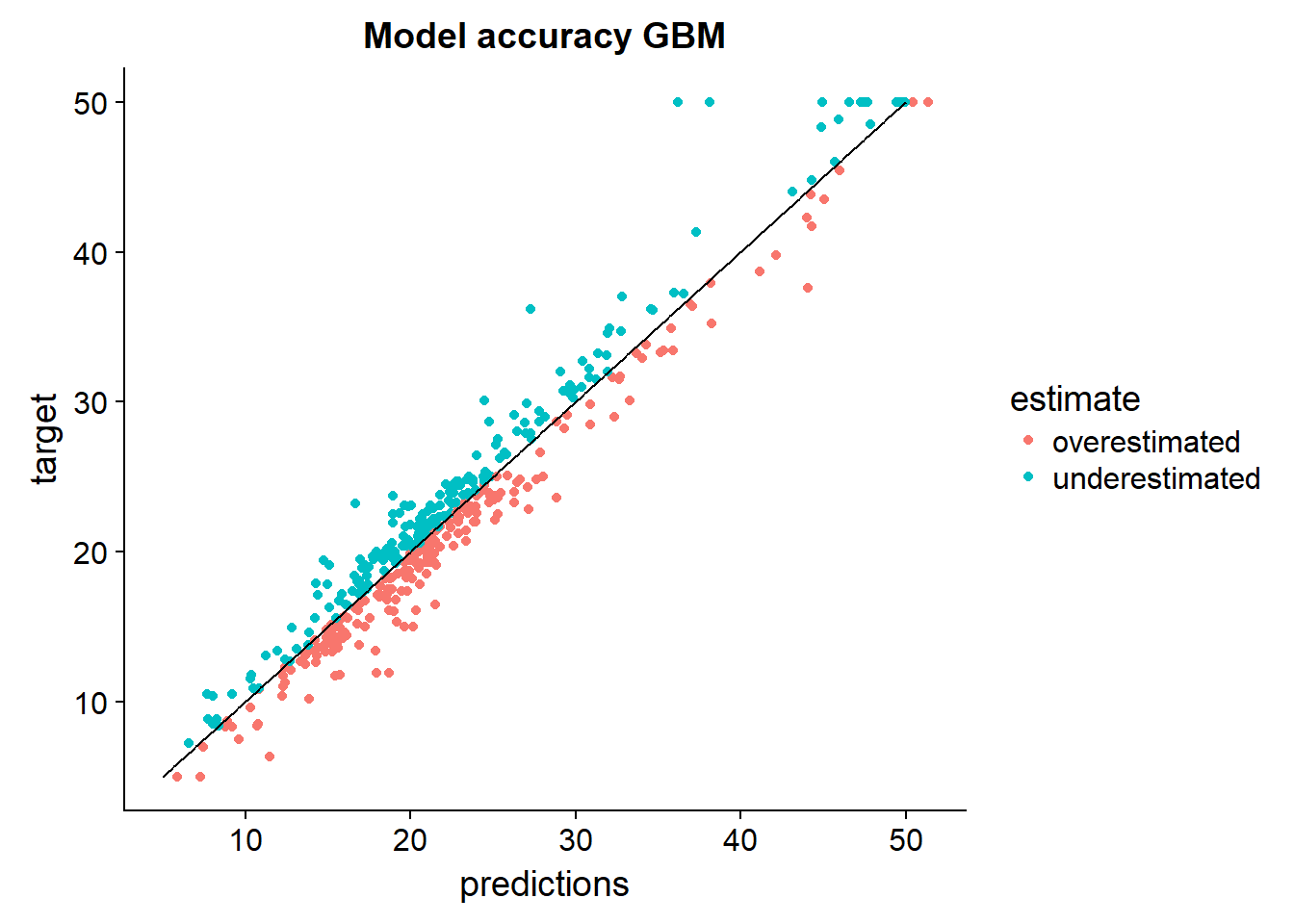What can we say about the bias of the model:

output_data %>%
arrange(target) %>%
mutate(perfect = seq(5,50,length.out = nrow(.))) %>%
mutate(estimate = ifelse(target > predictions,"underestimated","overestimated")) %>%
select(predictions,target,estimate,perfect) %>%
group_by(estimate) %>%
tally %>%
ggplot()+
geom_bar(aes(x= estimate, y=n, fill = estimate),stat="identity")+
ggtitle("GBM has low model error and less bias")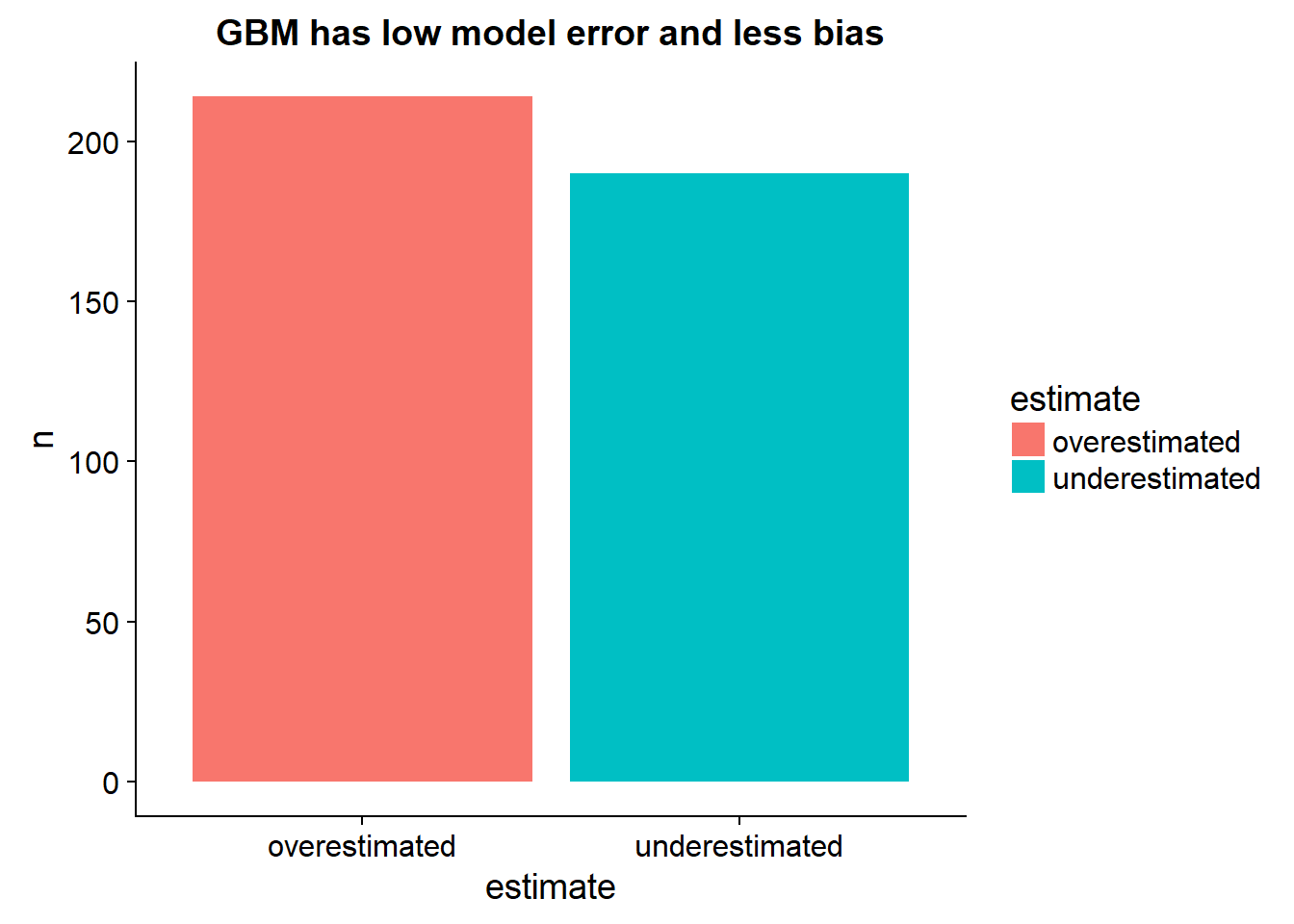Time series models using LSTM together with an inference network

Besides a dense feedforward network we can also predict time series movements of house prices using a LSTM network. This will allow us to predict the average move in house prices over the next couple of months

All residential home sales in Ames, Iowa between 2006 and 2010

# https://www.openintro.org/stat/data/ames.csv

if(!file.exists("../../static/data/cencus_data.csv")){
}

So we can tell that in this data we have primarily just a time index and a value. This is enough for us but we need to pre-process and label these 2 fields in a tidy dataframe before we start ironing out the model process.

housing_time_series <-

##    Order       PID MS.SubClass MS.Zoning Lot.Frontage Lot.Area Street
## 1:     1 526301100          20        RL          141    31770   Pave
## 2:     2 526350040          20        RH           80    11622   Pave
## 3:     3 526351010          20        RL           81    14267   Pave
## 4:     4 526353030          20        RL           93    11160   Pave
## 5:     5 527105010          60        RL           74    13830   Pave
## 6:     6 527105030          60        RL           78     9978   Pave
##    Alley Lot.Shape Land.Contour Utilities Lot.Config Land.Slope
## 1:    NA       IR1          Lvl    AllPub     Corner        Gtl
## 2:    NA       Reg          Lvl    AllPub     Inside        Gtl
## 3:    NA       IR1          Lvl    AllPub     Corner        Gtl
## 4:    NA       Reg          Lvl    AllPub     Corner        Gtl
## 5:    NA       IR1          Lvl    AllPub     Inside        Gtl
## 6:    NA       IR1          Lvl    AllPub     Inside        Gtl
##    Neighborhood Condition.1 Condition.2 Bldg.Type House.Style Overall.Qual
## 1:        NAmes        Norm        Norm      1Fam      1Story            6
## 2:        NAmes       Feedr        Norm      1Fam      1Story            5
## 3:        NAmes        Norm        Norm      1Fam      1Story            6
## 4:        NAmes        Norm        Norm      1Fam      1Story            7
## 5:      Gilbert        Norm        Norm      1Fam      2Story            5
## 6:      Gilbert        Norm        Norm      1Fam      2Story            6
##    Overall.Cond Year.Built Year.Remod.Add Roof.Style Roof.Matl
## 1:            5       1960           1960        Hip   CompShg
## 2:            6       1961           1961      Gable   CompShg
## 3:            6       1958           1958        Hip   CompShg
## 4:            5       1968           1968        Hip   CompShg
## 5:            5       1997           1998      Gable   CompShg
## 6:            6       1998           1998      Gable   CompShg
##    Exterior.1st Exterior.2nd Mas.Vnr.Type Mas.Vnr.Area Exter.Qual
## 1:      BrkFace      Plywood        Stone          112         TA
## 2:      VinylSd      VinylSd         None            0         TA
## 3:      Wd Sdng      Wd Sdng      BrkFace          108         TA
## 4:      BrkFace      BrkFace         None            0         Gd
## 5:      VinylSd      VinylSd         None            0         TA
## 6:      VinylSd      VinylSd      BrkFace           20         TA
##    Exter.Cond Foundation Bsmt.Qual Bsmt.Cond Bsmt.Exposure BsmtFin.Type.1
## 1:         TA     CBlock        TA        Gd            Gd            BLQ
## 2:         TA     CBlock        TA        TA            No            Rec
## 3:         TA     CBlock        TA        TA            No            ALQ
## 4:         TA     CBlock        TA        TA            No            ALQ
## 5:         TA      PConc        Gd        TA            No            GLQ
## 6:         TA      PConc        TA        TA            No            GLQ
##    BsmtFin.SF.1 BsmtFin.Type.2 BsmtFin.SF.2 Bsmt.Unf.SF Total.Bsmt.SF
## 1:          639            Unf            0         441          1080
## 2:          468            LwQ          144         270           882
## 3:          923            Unf            0         406          1329
## 4:         1065            Unf            0        1045          2110
## 5:          791            Unf            0         137           928
## 6:          602            Unf            0         324           926
##    Heating Heating.QC Central.Air Electrical X1st.Flr.SF X2nd.Flr.SF
## 1:    GasA         Fa           Y      SBrkr        1656           0
## 2:    GasA         TA           Y      SBrkr         896           0
## 3:    GasA         TA           Y      SBrkr        1329           0
## 4:    GasA         Ex           Y      SBrkr        2110           0
## 5:    GasA         Gd           Y      SBrkr         928         701
## 6:    GasA         Ex           Y      SBrkr         926         678
##    Low.Qual.Fin.SF Gr.Liv.Area Bsmt.Full.Bath Bsmt.Half.Bath Full.Bath
## 1:               0        1656              1              0         1
## 2:               0         896              0              0         1
## 3:               0        1329              0              0         1
## 4:               0        2110              1              0         2
## 5:               0        1629              0              0         2
## 6:               0        1604              0              0         2
##    Half.Bath Bedroom.AbvGr Kitchen.AbvGr Kitchen.Qual TotRms.AbvGrd
## 1:         0             3             1           TA             7
## 2:         0             2             1           TA             5
## 3:         1             3             1           Gd             6
## 4:         1             3             1           Ex             8
## 5:         1             3             1           TA             6
## 6:         1             3             1           Gd             7
##    Functional Fireplaces Fireplace.Qu Garage.Type Garage.Yr.Blt
## 1:        Typ          2           Gd      Attchd          1960
## 2:        Typ          0           NA      Attchd          1961
## 3:        Typ          0           NA      Attchd          1958
## 4:        Typ          2           TA      Attchd          1968
## 5:        Typ          1           TA      Attchd          1997
## 6:        Typ          1           Gd      Attchd          1998
##    Garage.Finish Garage.Cars Garage.Area Garage.Qual Garage.Cond
## 1:           Fin           2         528          TA          TA
## 2:           Unf           1         730          TA          TA
## 3:           Unf           1         312          TA          TA
## 4:           Fin           2         522          TA          TA
## 5:           Fin           2         482          TA          TA
## 6:           Fin           2         470          TA          TA
##    Paved.Drive Wood.Deck.SF Open.Porch.SF Enclosed.Porch X3Ssn.Porch
## 1:           P          210            62              0           0
## 2:           Y          140             0              0           0
## 3:           Y          393            36              0           0
## 4:           Y            0             0              0           0
## 5:           Y          212            34              0           0
## 6:           Y          360            36              0           0
##    Screen.Porch Pool.Area Pool.QC Fence Misc.Feature Misc.Val Mo.Sold
## 1:            0         0      NA    NA           NA        0       5
## 2:          120         0      NA MnPrv           NA        0       6
## 3:            0         0      NA    NA         Gar2    12500       6
## 4:            0         0      NA    NA           NA        0       4
## 5:            0         0      NA MnPrv           NA        0       3
## 6:            0         0      NA    NA           NA        0       6
##    Yr.Sold Sale.Type Sale.Condition SalePrice
## 1:    2010       WD          Normal    215000
## 2:    2010       WD          Normal    105000
## 3:    2010       WD          Normal    172000
## 4:    2010       WD          Normal    244000
## 5:    2010       WD          Normal    189900
## 6:    2010       WD          Normal    195500

Process data

We need to:
- Deal with NA’s
- Create vectorized tensors
- Scale tensors into small values
- Make sure all tensors have homogeneous scale
- Split data into time series and inference sets

We need to create a date formatted feature and we need to parse our categorical features to vectorized tensors. The reason we do this is because we want to fit 2 different models at the same time. One model will predict the price of a property based on the autocorrelations in the data, much like an arima or ets model would (we can use LSTM networks for this), and the other model will predict the price of the home given the 1D tensor data describing the property - street, size, zoning etc.

Let’s count NA’s

NA_info <-
housing_time_series %>%
as_tibble() %>%
map_df(~.x %>% is.na %>% sum) %>%
gather(key = "feature", value = "count_NA") %>%
arrange(-count_NA)

So out of the 82 columns in the data the colums that have more than 50 NA’s are

remove_features_str <-
NA_info %>%
filter(count_NA>50) %>%
pull(feature)

remove_features_str
##   "Pool.QC"        "Misc.Feature"   "Alley"          "Fence"
##   "Fireplace.Qu"   "Lot.Frontage"   "Garage.Yr.Blt"  "Garage.Qual"
##   "Garage.Cond"    "Garage.Type"    "Garage.Finish"  "Bsmt.Qual"
##  "Bsmt.Cond"      "Bsmt.Exposure"  "BsmtFin.Type.1" "BsmtFin.Type.2"
remove_features_str %>% length()
##  16

For the sake of this tutorial we will just throw these away but it may be useful to impute these using nearest neighbors or mean imputations. You can leverage something like the mice package.

Remove the columns and remove leftover NA observations

housing_time_series_cleaned <-
housing_time_series %>%
select(-which(names(.) %in% remove_features_str)) %>%
na.omit() %>%
select(SalePrice,Yr.Sold,Mo.Sold,everything()) %>%
select(-PID,-Order
)

housing_time_series_cleaned %>% dim()
##  2904   64

So what we are left with is a table with 66 features and 2904 samples…

Split the data for 2 models

We want to train a model to predict house prices using the descriptive features and also train an lstm model to predict future price movements.

First we need to split test train sets:

split_data <-
caret::createDataPartition(housing_time_series_cleaned$SalePrice, p = 0.8, list = FALSE) train <- housing_time_series_cleaned[split_data,] test <- housing_time_series_cleaned[-split_data,] It’s important that our time series does not have missing months since we will lag time vectors later on… If there were missing months the auto correlation of say n month lags will get mixed up with n+1 month lags. Let’s check this: interaction(test$Yr.Sold,test$Mo.Sold) %>% unique() ##  2010.5 2010.2 2010.1 2010.6 2010.4 2010.3 2010.7 2009.10 ##  2009.6 2009.5 2009.12 2009.7 2009.8 2009.3 2009.2 2009.9 ##  2009.11 2009.4 2009.1 2008.5 2008.8 2008.10 2008.7 2008.6 ##  2008.3 2008.11 2008.9 2008.2 2008.1 2008.4 2008.12 2007.5 ##  2007.8 2007.3 2007.7 2007.6 2007.10 2007.1 2007.9 2007.4 ##  2007.11 2007.12 2007.2 2006.8 2006.12 2006.9 2006.7 2006.1 ##  2006.3 2006.2 2006.6 2006.4 2006.11 2006.5 2006.10 ## 60 Levels: 2006.1 2007.1 2008.1 2009.1 2010.1 2006.2 2007.2 ... 2010.12 interaction(train$Yr.Sold,train$Mo.Sold) %>% unique() ##  2010.5 2010.6 2010.4 2010.3 2010.1 2010.2 2010.7 2009.7 ##  2009.8 2009.6 2009.10 2009.11 2009.9 2009.2 2009.12 2009.5 ##  2009.4 2009.3 2009.1 2008.5 2008.6 2008.4 2008.10 2008.7 ##  2008.11 2008.8 2008.9 2008.3 2008.12 2008.1 2008.2 2007.3 ##  2007.4 2007.2 2007.10 2007.7 2007.8 2007.6 2007.5 2007.9 ##  2007.11 2007.1 2007.12 2006.5 2006.6 2006.10 2006.3 2006.11 ##  2006.2 2006.7 2006.1 2006.9 2006.8 2006.12 2006.4 ## 60 Levels: 2006.1 2007.1 2008.1 2009.1 2010.1 2006.2 2007.2 ... 2010.12 Since both sets have all 60 months we don’t have any problems here… For the LSTM model we need only the time index and the value: For the time series model we are going to train on the moving average monthly house price so the complete model can use this in predictions LSTM_train <- train %>% select(SalePrice,Yr.Sold,Mo.Sold) %>% tidyr::unite(col = index,Yr.Sold,Mo.Sold,sep="-") %>% mutate(index = index %>% zoo::as.yearmon() %>% as_date()) %>% rename(value = SalePrice) %>% group_by(index) %>% summarise(value = mean(value,na.rm = TRUE)) %>% as_tbl_time(index = index) LSTM_test <- test %>% select(SalePrice,Yr.Sold,Mo.Sold) %>% tidyr::unite(col = index,Yr.Sold,Mo.Sold,sep="-") %>% mutate(index = index %>% zoo::as.yearmon() %>% as_date()) %>% rename(value = SalePrice) %>% group_by(index) %>% summarise(value = mean(value,na.rm = TRUE)) %>% as_tbl_time(index = index) LSTM_train %>% head ## # A time tibble: 6 x 2 ## # Index: index ## index value ## <date> <dbl> ## 1 2006-01-01 212711. ## 2 2006-02-01 182493. ## 3 2006-03-01 180162. ## 4 2006-04-01 170443. ## 5 2006-05-01 170079. ## 6 2006-06-01 178162. Function that one_hot encodes classes for prediction one_hot_categorical_inputs_fn <- function(tibble_df) { one_hot_list <- tibble_df %>% map_if(is.character,as_factor) %>% map_if(is.factor,keras::to_categorical) bind_binary_classes <- one_hot_list %>% purrr::keep(is.matrix) %>% reduce(cbind) bind_numeric_features <- one_hot_list %>% purrr::discard(is.matrix) %>% reduce(cbind) %>% as.matrix() return(cbind(bind_numeric_features,bind_binary_classes)) } Before we cast everything to numeric we should scale these numeric variables since the new sparse matrices will have 1’s and 0’s: Function that will scale all the numeric variables scale_numerics_fn <- function(df_in, mean = NULL, std = NULL,...) { numeric_cols <- df_in %>% map_lgl(is.numeric) scaled_numeric <- df_in %>% select_if(numeric_cols) if(is.null(mean) | is.null(std)) { mean <- apply(scaled_numeric, 2, mean) std <- apply(scaled_numeric, 2, sd) } recombined <- cbind( scale(scaled_numeric, center = mean, scale = std), df_in %>% select_if(!numeric_cols) ) return(list(recombined = recombined,mean = mean, std = std)) } Scale numerics… (Remember that the test set must be scaled using the training scales otherwise you wouldn’t be predicting using the same data scale as trained) Here we will start out with the original cleaned data again because we want to spread the same number of classes in the train and test sets data_scaled <- housing_time_series_cleaned %>% select(-Yr.Sold,-Mo.Sold,-SalePrice) data_scaled_outp <- data_scaled %>% scale_numerics_fn data_scaled <- data_scaled_outp$recombined

inference_X <-
data_scaled %>%
one_hot_categorical_inputs_fn()

inference_target <-
housing_time_series_cleaned %>%
select(SalePrice) %>%
as.matrix()

##              out
## [1,] -0.87715441  2.74965990 -0.06154026 -0.5089121 -0.3685560 -1.1560238
## [2,] -0.87715441  0.18952801 -0.77071847  0.3889498 -0.3354663 -1.1080859
## [3,] -0.87715441  0.52561839 -0.06154026  0.3889498 -0.4347354 -1.2518997
## [4,] -0.87715441  0.13082338  0.64763795 -0.5089121 -0.1038383 -0.7725204
## [5,]  0.05903575  0.47009041 -0.77071847 -0.5089121  0.8557633  0.6656175
## [6,]  0.05903575 -0.01936899 -0.06154026  0.3889498  0.8888530  0.6656175
##
## [1,]  0.05579978 0.43144278 -0.2947952 -0.2670621  0.06612207  1.2816766
## [2,] -0.56928686 0.05583144  0.5533971 -0.6565320 -0.38356992 -0.6750520
## [3,]  0.03347525 1.05526512 -0.2947952 -0.3467782  0.63164381  0.4397683
## [4,] -0.56928686 1.36717629 -0.2947952  1.1086092  2.40542888  2.4505644
## [5,] -0.56928686 0.76531953 -0.2947952 -0.9594530 -0.27909603 -0.5926635
## [6,] -0.45766424 0.35017015 -0.2947952 -0.5335415 -0.28363837 -0.5978128
##
## [1,] -0.7820247 -0.1014432  0.3137316  1.0827555 -0.2503599 -1.019769
## [2,] -0.7820247 -0.1014432 -1.1925373 -0.8217399 -0.2503599 -1.019769
## [3,] -0.7820247 -0.1014432 -0.3343604 -0.8217399 -0.2503599 -1.019769
## [4,] -0.7820247 -0.1014432  1.2135291  1.0827555 -0.2503599  0.793709
## [5,]  0.8554028 -0.1014432  0.2602194 -0.8217399 -0.2503599  0.793709
## [6,]  0.8016783 -0.1014432  0.2106711 -0.8217399 -0.2503599  0.793709
##
## [1,] -0.7518758  0.1759575 -0.2073247  0.3546094  2.1638512  0.3093533
## [2,] -0.7518758 -1.0320345 -0.2073247 -0.9167310 -0.9240229 -1.0040415
## [3,]  1.2421400  0.1759575 -0.2073247 -0.2810608 -0.9240229 -1.0040415
## [4,]  1.2421400  0.1759575 -0.2073247  0.9902796  2.1638512  0.3093533
## [5,]  1.2421400  0.1759575 -0.2073247 -0.2810608  0.6199141  0.3093533
## [6,]  1.2421400  0.1759575 -0.2073247  0.3546094  0.6199141  0.3093533
##
## [1,]  0.2588252  0.9160874  0.2215652 -0.3587571 -0.1035821 -0.2866842
## [2,]  1.1972919  0.3632432 -0.7032610 -0.3587571 -0.1035821  1.8440946
## [3,] -0.7446839  2.3613800 -0.1662651 -0.3587571 -0.1035821 -0.2866842
## [4,]  0.2309499 -0.7424451 -0.7032610 -0.3587571 -0.1035821 -0.2866842
## [5,]  0.0451149  0.9318829 -0.1960982 -0.3587571 -0.1035821 -0.2866842
## [6,] -0.0106356  2.1007535 -0.1662651 -0.3587571 -0.1035821 -0.2866842
##
## [1,] -0.06330281 -0.08980944 0 1 0 0 0 0 0 0 0 1 0 0 1 0 0 0 0 1 0 0 0 0 1
## [2,] -0.06330281 -0.08980944 0 0 1 0 0 0 0 0 0 1 0 0 0 1 0 0 0 1 0 0 0 0 1
## [3,] -0.06330281 21.88417774 0 1 0 0 0 0 0 0 0 1 0 0 1 0 0 0 0 1 0 0 0 0 1
## [4,] -0.06330281 -0.08980944 0 1 0 0 0 0 0 0 0 1 0 0 0 1 0 0 0 1 0 0 0 0 1
## [5,] -0.06330281 -0.08980944 0 1 0 0 0 0 0 0 0 1 0 0 1 0 0 0 0 1 0 0 0 0 1
## [6,] -0.06330281 -0.08980944 0 1 0 0 0 0 0 0 0 1 0 0 1 0 0 0 0 1 0 0 0 0 1
##
## [1,] 0 0 0 1 0 0 0 0 0 1 0 0 0 1 0 0 0 0 0 0 0 0 0 0 0 0 0 0 0 0 0 0 0 0 0
## [2,] 0 0 0 0 1 0 0 0 0 1 0 0 0 1 0 0 0 0 0 0 0 0 0 0 0 0 0 0 0 0 0 0 0 0 0
## [3,] 0 0 0 1 0 0 0 0 0 1 0 0 0 1 0 0 0 0 0 0 0 0 0 0 0 0 0 0 0 0 0 0 0 0 0
## [4,] 0 0 0 1 0 0 0 0 0 1 0 0 0 1 0 0 0 0 0 0 0 0 0 0 0 0 0 0 0 0 0 0 0 0 0
## [5,] 0 0 0 0 1 0 0 0 0 1 0 0 0 0 1 0 0 0 0 0 0 0 0 0 0 0 0 0 0 0 0 0 0 0 0
## [6,] 0 0 0 0 1 0 0 0 0 1 0 0 0 0 1 0 0 0 0 0 0 0 0 0 0 0 0 0 0 0 0 0 0 0 0
##
## [1,] 0 0 0 0 0 0 0 1 0 0 0 0 0 0 0 0 0 1 0 0 0 0 0 0 0 0 1 0 0 0 0 0 1 0 0
## [2,] 0 0 0 0 0 0 0 0 1 0 0 0 0 0 0 0 0 1 0 0 0 0 0 0 0 0 1 0 0 0 0 0 1 0 0
## [3,] 0 0 0 0 0 0 0 1 0 0 0 0 0 0 0 0 0 1 0 0 0 0 0 0 0 0 1 0 0 0 0 0 1 0 0
## [4,] 0 0 0 0 0 0 0 1 0 0 0 0 0 0 0 0 0 1 0 0 0 0 0 0 0 0 1 0 0 0 0 0 1 0 0
## [5,] 0 0 0 0 0 0 0 1 0 0 0 0 0 0 0 0 0 1 0 0 0 0 0 0 0 0 1 0 0 0 0 0 0 1 0
## [6,] 0 0 0 0 0 0 0 1 0 0 0 0 0 0 0 0 0 1 0 0 0 0 0 0 0 0 1 0 0 0 0 0 0 1 0
##
## [1,] 0 0 0 0 0 0 1 0 0 0 0 0 0 1 0 0 0 0 0 0 0 0 1 0 0 0 0 0 0 0 0 0 0 0 0
## [2,] 0 0 0 0 0 0 0 1 0 0 0 0 0 1 0 0 0 0 0 0 0 0 0 1 0 0 0 0 0 0 0 0 0 0 0
## [3,] 0 0 0 0 0 0 1 0 0 0 0 0 0 1 0 0 0 0 0 0 0 0 0 0 1 0 0 0 0 0 0 0 0 0 0
## [4,] 0 0 0 0 0 0 1 0 0 0 0 0 0 1 0 0 0 0 0 0 0 0 1 0 0 0 0 0 0 0 0 0 0 0 0
## [5,] 0 0 0 0 0 0 0 1 0 0 0 0 0 1 0 0 0 0 0 0 0 0 0 1 0 0 0 0 0 0 0 0 0 0 0
## [6,] 0 0 0 0 0 0 0 1 0 0 0 0 0 1 0 0 0 0 0 0 0 0 0 1 0 0 0 0 0 0 0 0 0 0 0
##
## [1,] 0 0 0 0 1 0 0 0 0 0 0 0 0 0 0 0 0 0 0 0 0 0 1 0 0 0 0 0 1 0 0 0 0 1 0
## [2,] 0 0 0 0 0 1 0 0 0 0 0 0 0 0 0 0 0 0 0 0 0 0 0 1 0 0 0 0 1 0 0 0 0 1 0
## [3,] 0 0 0 0 0 0 1 0 0 0 0 0 0 0 0 0 0 0 0 0 0 0 0 0 1 0 0 0 1 0 0 0 0 1 0
## [4,] 0 0 0 0 0 0 0 1 0 0 0 0 0 0 0 0 0 0 0 0 0 0 0 1 0 0 0 0 0 1 0 0 0 1 0
## [5,] 0 0 0 0 0 1 0 0 0 0 0 0 0 0 0 0 0 0 0 0 0 0 0 1 0 0 0 0 1 0 0 0 0 1 0
## [6,] 0 0 0 0 0 1 0 0 0 0 0 0 0 0 0 0 0 0 0 0 0 0 0 0 1 0 0 0 1 0 0 0 0 1 0
##
## [1,] 0 0 0 0 1 0 0 0 0 0 0 1 0 0 0 0 0 0 1 0 0 0 0 0 1 0 0 1 0 0 0 0 0 0 1
## [2,] 0 0 0 0 1 0 0 0 0 0 0 1 0 0 0 0 0 0 0 1 0 0 0 0 1 0 0 1 0 0 0 0 0 0 1
## [3,] 0 0 0 0 1 0 0 0 0 0 0 1 0 0 0 0 0 0 0 1 0 0 0 0 1 0 0 1 0 0 0 0 0 0 0
## [4,] 0 0 0 0 1 0 0 0 0 0 0 1 0 0 0 0 0 0 0 0 1 0 0 0 1 0 0 1 0 0 0 0 0 0 0
## [5,] 0 0 0 0 0 1 0 0 0 0 0 1 0 0 0 0 0 0 0 0 0 1 0 0 1 0 0 1 0 0 0 0 0 0 1
## [6,] 0 0 0 0 0 1 0 0 0 0 0 1 0 0 0 0 0 0 0 0 1 0 0 0 1 0 0 1 0 0 0 0 0 0 0
##
## [1,] 0 0 0 0 0 1 0 0 0 0 0 0 0 0 1 0 0 0 1 0 0 0 0 0 0 0 0 0 0 1 0 0 0 0 0
## [2,] 0 0 0 0 0 1 0 0 0 0 0 0 0 0 0 1 0 0 1 0 0 0 0 0 0 0 0 0 0 1 0 0 0 0 0
## [3,] 1 0 0 0 0 1 0 0 0 0 0 0 0 0 0 1 0 0 1 0 0 0 0 0 0 0 0 0 0 1 0 0 0 0 0
## [4,] 0 1 0 0 0 1 0 0 0 0 0 0 0 0 0 1 0 0 1 0 0 0 0 0 0 0 0 0 0 1 0 0 0 0 0
## [5,] 0 0 0 0 0 1 0 0 0 0 0 0 0 0 0 1 0 0 1 0 0 0 0 0 0 0 0 0 0 1 0 0 0 0 0
## [6,] 1 0 0 0 0 1 0 0 0 0 0 0 0 0 0 1 0 0 1 0 0 0 0 0 0 0 0 0 0 1 0 0 0 0 0
##      SalePrice
## [1,]    215000
## [2,]    105000
## [3,]    172000
## [4,]    244000
## [5,]    189900
## [6,]    195500
inference_train_X <-
inference_X[split_data,]

inference_test_X <-
inference_X[-split_data,]

inference_train_target <-
inference_target[split_data,]

inference_test_target <-
inference_target[-split_data,]

So we have 265 dimesnions on the tensor axis

So at this point we have nicely scaled and homogeneous inputs in a nice vectorized format for our tensors to learn with. The only data we have not vectorized is the time series data we will use in the LSTM modelNow that our data is processed we can try to build the skeleton of our inference model that we will train together with the LSTM model

We can use the same structure we used in the naive model:

define_compile_train_predict <- function(train_X,test_X,train_y,test_y,epochs = 100,shuffle = TRUE,...){

#define
base_model <-
keras_model_sequential() %>%
layer_dense(units = 128, activation = 'relu', input_shape = dim(train_X)) %>% # 1 axis and nr features = number columns
layer_batch_normalization() %>%
# layer_dropout(rate = 0.2) %>%
layer_dense(units = 64, activation ='relu') %>%
layer_dense(units = 32, activation ='relu') %>%
# layer_dense(units = 25, activation ='relu') %>%
layer_dense(units = 1)

#compile
base_model %>% compile(
loss='mse',
optimizer='rmsprop',
metrics = c('mae')
)

#train
history <- base_model %>%
keras::fit(train_X,
train_y,
epochs=epochs,
shuffle=shuffle,
validation_data= list(test_X, test_y),
verbose = 0
)

#predict
predictions <-
base_model %>%
keras::predict_on_batch(x = train_X)

results <-
base_model %>%
keras::evaluate(test_X, test_y, verbose = 0)

plot_outp <-
history %>% plot

return(list(predictions = predictions, loss = results$loss, mae = results$mean_absolute_error, plot_outp = plot_outp,base_model=base_model,history = history))

}

Let’s see out of box performance of our inference network:

model_outp <-
define_compile_train_predict(train_X = inference_train_X, train_y = inference_train_target,test_X = inference_test_X, test_y = inference_test_target)

model_outp$plot_outp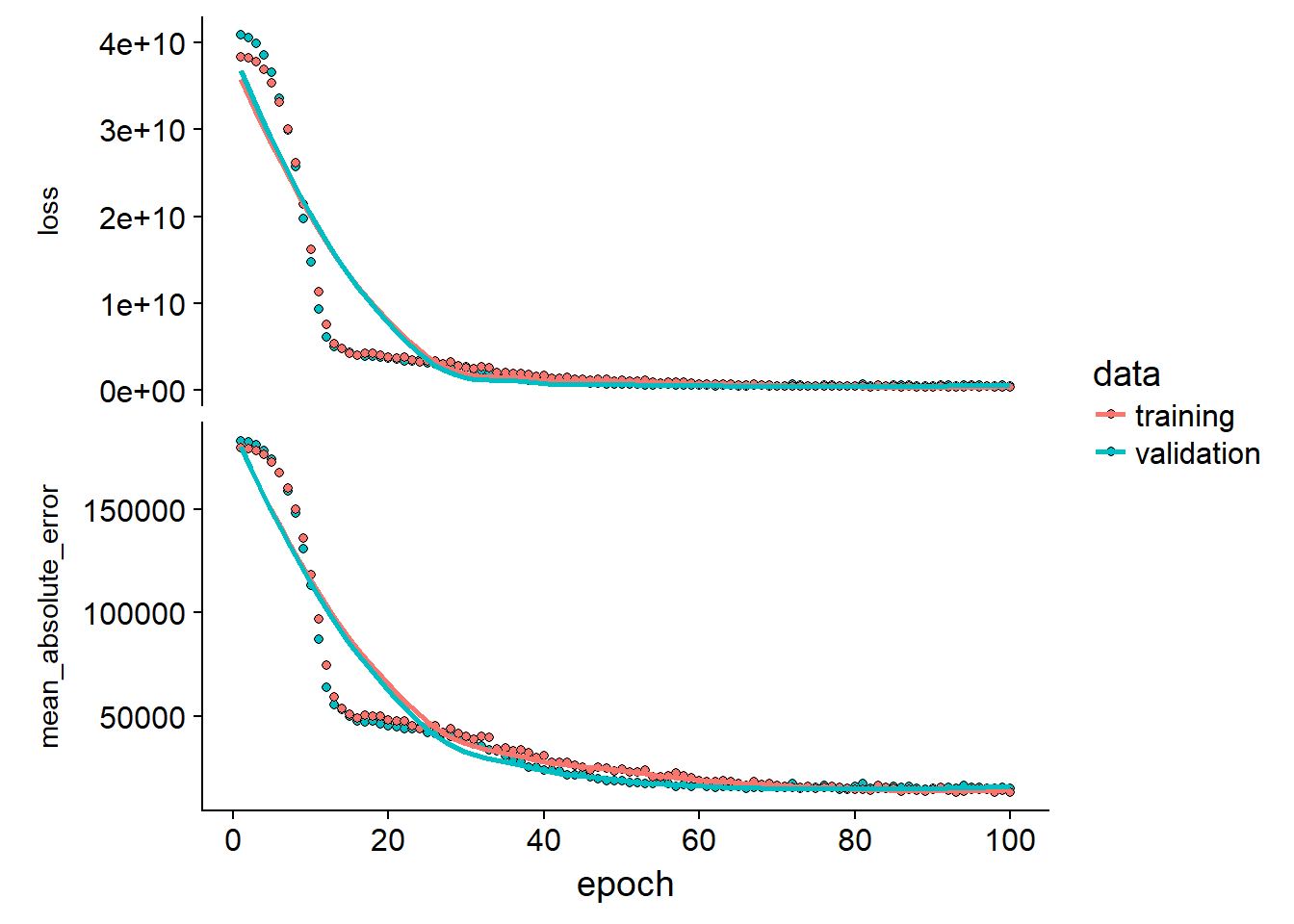model_outp$history$metrics$val_mean_absolute_error %>% last()
##  14664.32

This looks promising… If we calculate the MAE / average price: 8.1628129 %

This is the expected % error before we optimize the model and combine the LSTM network. I have not yet tested and tuned the network here so with some revision to the network we could potentially improve this by a lot.

Design LSTM model

Let’s start by visualizing the time series data…

Now remember we made the training time tibble:

## # A time tibble: 6 x 2
## # Index: index
##   index        value
##   <date>       <dbl>
## 1 2006-01-01 212711.
## 2 2006-02-01 182493.
## 3 2006-03-01 180162.
## 4 2006-04-01 170443.
## 5 2006-05-01 170079.
## 6 2006-06-01 178162.

Let’s visualize the average price

LSTM_train %>%
# filter_time("2008" ~ "end") %>%
ggplot(aes(index, value)) +
geom_line(color = palette_light()[], alpha = 0.5) +
geom_point(color = palette_light()[]) +
geom_smooth(method = "loess", span = 0.2, se = FALSE) +
theme_tq()+
labs(title = 'All residential home sales in Ames, Iowa between 2006 and 2010')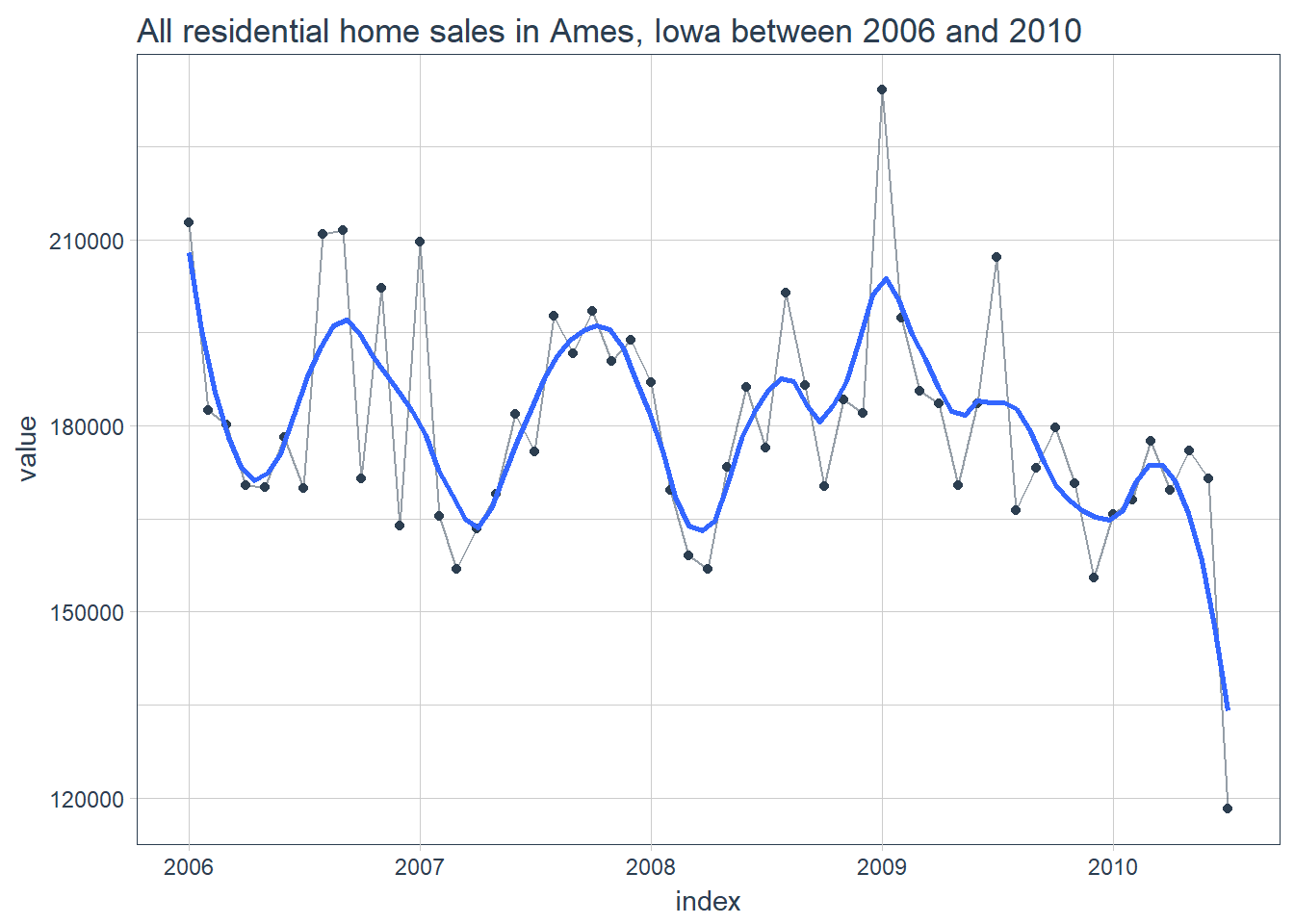So this timeseries actually looks surprisingly stationary with expected volatility around the 2009 market crash. We will have to see if a LSTM network can make some sense out of this.

We define the handy tidy_acf function

tidy_acf <- function(data, value, lags = 0:20) {

value_expr <- enquo(value)

acf_values <- data %>%
pull(value) %>%
acf(lag.max = tail(lags, 1), plot = FALSE) %>%
.$acf %>% .[,,1] ret <- tibble(acf = acf_values) %>% rowid_to_column(var = "lag") %>% mutate(lag = lag - 1) %>% filter(lag %in% lags) return(ret) } tidy_pacf <- function(data, value, lags = 0:20) { value_expr <- enquo(value) pacf_values <- data %>% pull(value) %>% pacf(lag.max = tail(lags, 1), plot = FALSE) %>% .$acf %>%
.[,,1]

ret <- tibble(pacf = pacf_values) %>%
rowid_to_column(var = "lag") %>%
mutate(lag = lag - 1) %>%
filter(lag %in% lags)

return(ret)
}

Let’s view the significant lags in the ACF and PACF

max_lag <- 100 # these are months, since our samples are sales in a month

LSTM_train %>%
tidy_acf(value, lags = 0:max_lag)
## # A tibble: 55 x 2
##      lag       acf
##    <dbl>     <dbl>
##  1    0.  1.00
##  2    1.  0.205
##  3    2.  0.148
##  4    3. -0.000328
##  5    4. -0.0990
##  6    5.  0.0441
##  7    6. -0.0976
##  8    7.  0.0221
##  9    8. -0.0316
## 10    9. -0.124
## # ... with 45 more rows
LSTM_train %>%
tidy_pacf(value, lags = 0:max_lag)
## # A tibble: 54 x 2
##      lag    pacf
##    <dbl>   <dbl>
##  1    0.  0.205
##  2    1.  0.111
##  3    2. -0.0529
##  4    3. -0.112
##  5    4.  0.0965
##  6    5. -0.101
##  7    6.  0.0382
##  8    7. -0.0279
##  9    8. -0.119
## 10    9.  0.0262
## # ... with 44 more rows

Visualize it:

LSTM_train %>%
tidy_acf(value, lags = 0:max_lag) %>%
ggplot(aes(lag, acf)) +
geom_segment(aes(xend = lag, yend = 0), color = palette_light()[]) +
geom_vline(xintercept = 2, size = 1, color = palette_light()[],alpha = 0.3) +
# geom_vline(xintercept = 25, size = 2, color = palette_light()[]) +
# annotate("text", label = "10 Year Mark", x = 130, y = 0.8,
# color = palette_light()[], size = 6, hjust = 0) +
theme_tq() +
labs(title = "ACF: Ames, Iowa home sales",subtitle = 'Mostly white noise, possible 2 lag')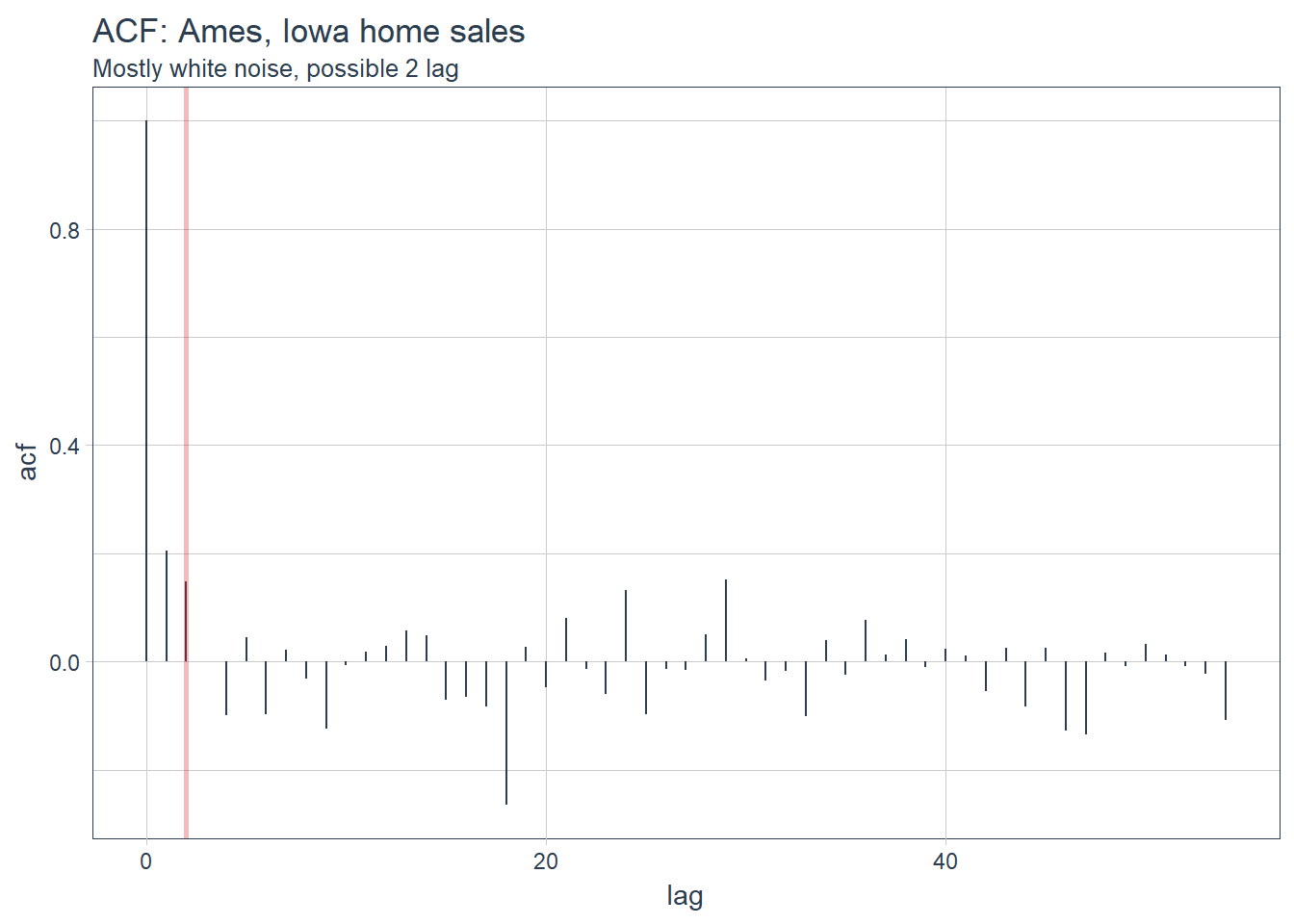LSTM_train %>%
tidy_pacf(value, lags = 0:max_lag) %>%
ggplot(aes(lag, pacf)) +
geom_segment(aes(xend = lag, yend = 0), color = palette_light()[]) +
# geom_vline(xintercept = 3, size = 1, color = palette_light()[],alpha = 0.3) +
# annotate("text", label = "10 Year Mark", x = 130, y = 0.8,
# color = palette_light()[], size = 6, hjust = 0) +
theme_tq() +
labs(title = "PACF: Ames, Iowa home sales",subtitle = 'Mostly white noise, possible 2 lag')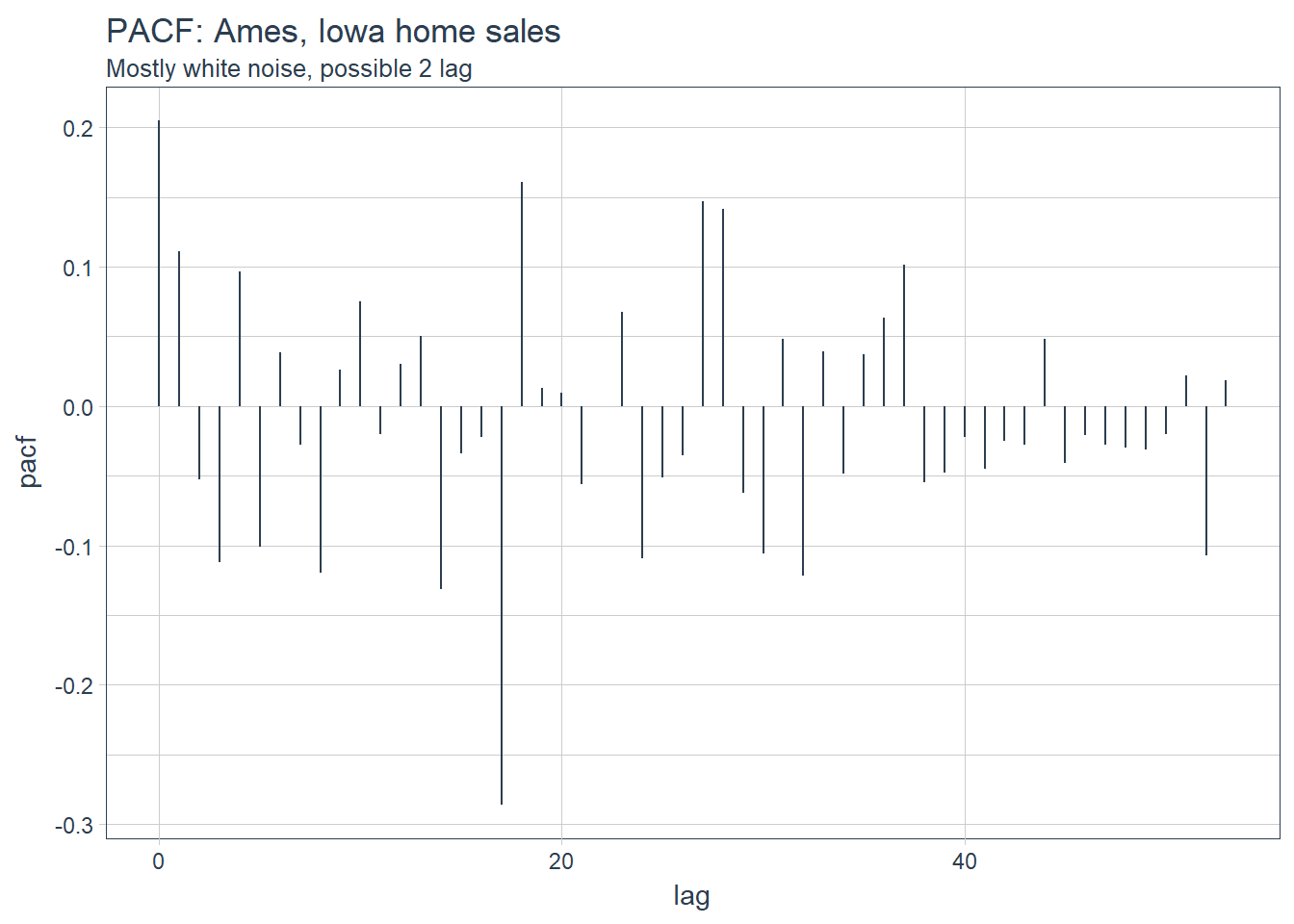To remain completely objective here we can consult the forecast package:

forecast::auto.arima(LSTM_train$value,max.q = 0) ## Series: LSTM_train$value
## ARIMA(1,0,0) with non-zero mean
##
## Coefficients:
##          ar1        mean
##       0.2717  179891.444
## s.e.  0.1504    3282.577
##
## sigma^2 estimated as 3.3e+08:  log likelihood=-616.46
## AIC=1238.92   AICc=1239.39   BIC=1244.94

For the sake of exploration we can try the ar 2 framework

LSTM_train %>%
tidy_acf(value, lags = 0:15) %>%
ggplot(aes(lag, acf)) +
geom_vline(xintercept = 2, size = 3, color = palette_light()[],alpha=0.3) +
geom_segment(aes(xend = lag, yend = 0), color = palette_light()[]) +
geom_point(color = palette_light()[], size = 2) +
geom_label(aes(label = acf %>% round(2)), vjust = -1,
color = palette_light()[]) +
annotate("text", label = "2 Month Mark", x = 3, y = 0.8,
color = palette_light()[], size = 5, hjust = 0) +
theme_tq() +
labs(title = "ACF: Iowa",
subtitle = "Zoomed in on Lags 1 to 15")optimal_lag_setting <- 2

For us to validate the model we will use a method called backtesting. Backtesting is basically splitting the data into different splits of data and for each split leaving out a future horizon that you can predict and measure accuracy. By testing all the different folds we can feel more certain of model performance since it wasn’t just a one time lucky score.

# periods_train <- 12 * 50
# periods_test  <- 12 * 10
# skip_span     <- 12 * 20

periods_train <- 36
periods_test  <- 3
skip_span     <- 6

rolling_origin_resamples <- rolling_origin(
LSTM_train,
initial    = periods_train,
assess     = periods_test,
cumulative = FALSE,
skip       = skip_span
)

rolling_origin_resamples
## # Rolling origin forecast resampling
## # A tibble: 3 x 2
##   splits       id
##   <list>       <chr>
## 1 <S3: rsplit> Slice1
## 2 <S3: rsplit> Slice2
## 3 <S3: rsplit> Slice3

A function that visualizes the different times series splits:

# Plotting function for a single split
plot_split <- function(split, expand_y_axis = TRUE, alpha = 1, size = 1, base_size = 14) {

# Manipulate data
train_tbl <- training(split) %>%

test_tbl  <- testing(split) %>%

data_manipulated <- bind_rows(train_tbl, test_tbl) %>%
as_tbl_time(index = index) %>%
mutate(key = fct_relevel(key, "training", "testing"))

# Collect attributes
train_time_summary <- train_tbl %>%
tk_index() %>%
tk_get_timeseries_summary()

test_time_summary <- test_tbl %>%
tk_index() %>%
tk_get_timeseries_summary()

# Visualize
g <- data_manipulated %>%
ggplot(aes(x = index, y = value, color = key)) +
geom_line(size = size, alpha = alpha) +
theme_tq(base_size = base_size) +
scale_color_tq() +
labs(
title    = glue("Split: {split$id}"), subtitle = glue("{train_time_summary$start} to {test_time_summary$end}"), y = "", x = "" ) + theme(legend.position = "none") if (expand_y_axis) { sun_spots_time_summary <- sun_spots %>% tk_index() %>% tk_get_timeseries_summary() g <- g + scale_x_date(limits = c(sun_spots_time_summary$start,
sun_spots_time_summary$end)) } return(g) } Plotting function that we can map over all these splits # Plotting function that scales to all splits plot_sampling_plan <- function(sampling_tbl, expand_y_axis = TRUE, ncol = 3, alpha = 1, size = 1, base_size = 14, title = "Sampling Plan") { # Map plot_split() to sampling_tbl sampling_tbl_with_plots <- sampling_tbl %>% mutate(gg_plots = map(splits, plot_split, expand_y_axis = expand_y_axis, alpha = alpha, base_size = base_size)) # Make plots with cowplot plot_list <- sampling_tbl_with_plots$gg_plots

p_temp <- plot_list[] + theme(legend.position = "bottom")
legend <- get_legend(p_temp)

p_body  <- plot_grid(plotlist = plot_list, ncol = ncol)

p_title <- ggdraw() +
draw_label(title, size = 18, fontface = "bold", colour = palette_light()[])

g <- plot_grid(p_title, p_body, legend, ncol = 1, rel_heights = c(0.05, 1, 0.05))

return(g)

}

Let’s visualize our backtesting folds:

rolling_origin_resamples %>%
plot_sampling_plan(expand_y_axis = FALSE, ncol = 3, alpha = 1, size = 1, base_size = 10,
title = "Backtesting Strategy: Rolling Origin Sampling Plan")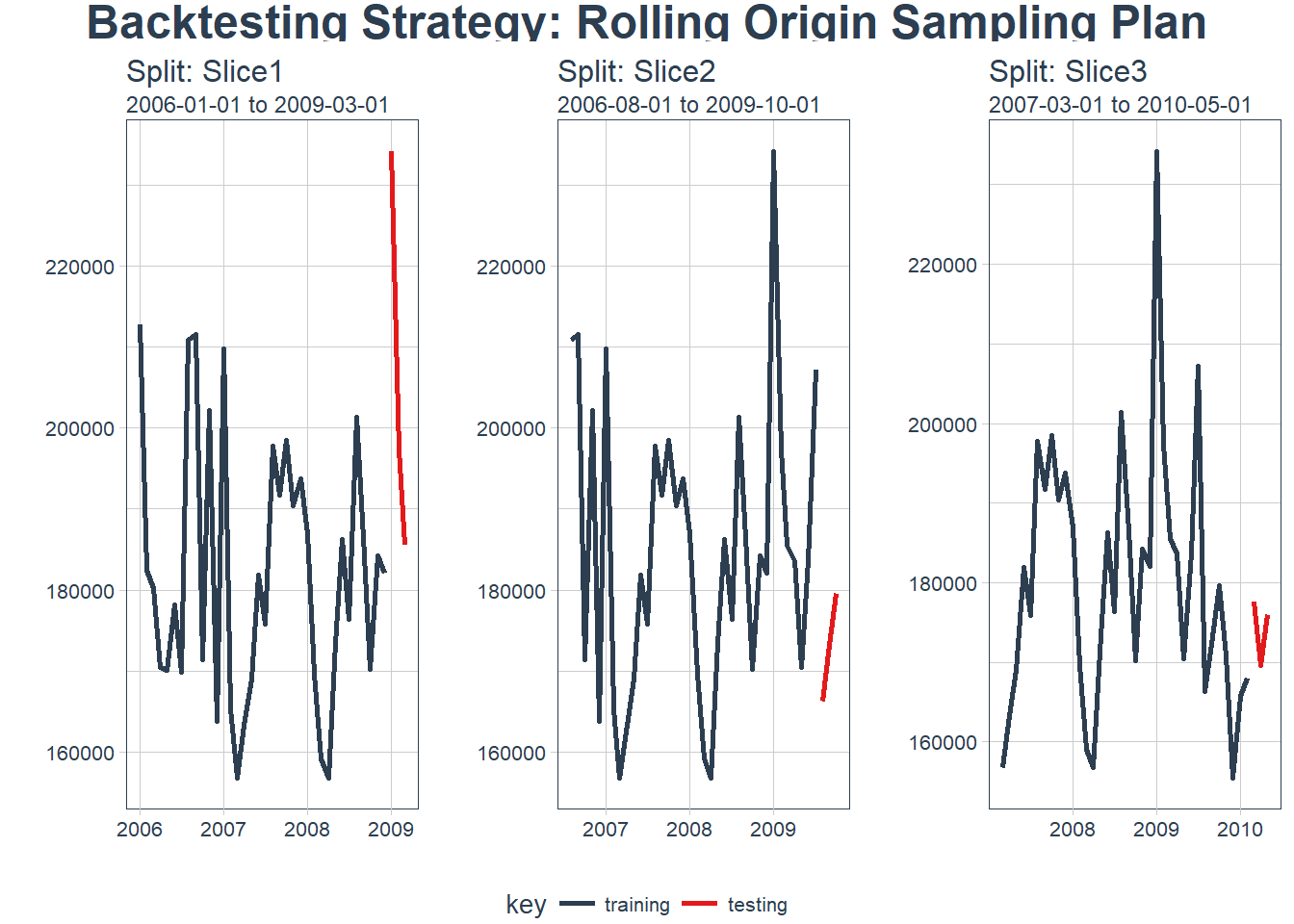Test a LSTM model

OK, so now that we have a backtesting strategy set we should try to fit a model on one of the folds:

split    <- rolling_origin_resamples$splits[] split_id <- rolling_origin_resamples$id[]

plot_split(split, expand_y_axis = FALSE, size = 0.5) +
theme(legend.position = "bottom") +
ggtitle(glue("Split: {split_id}"))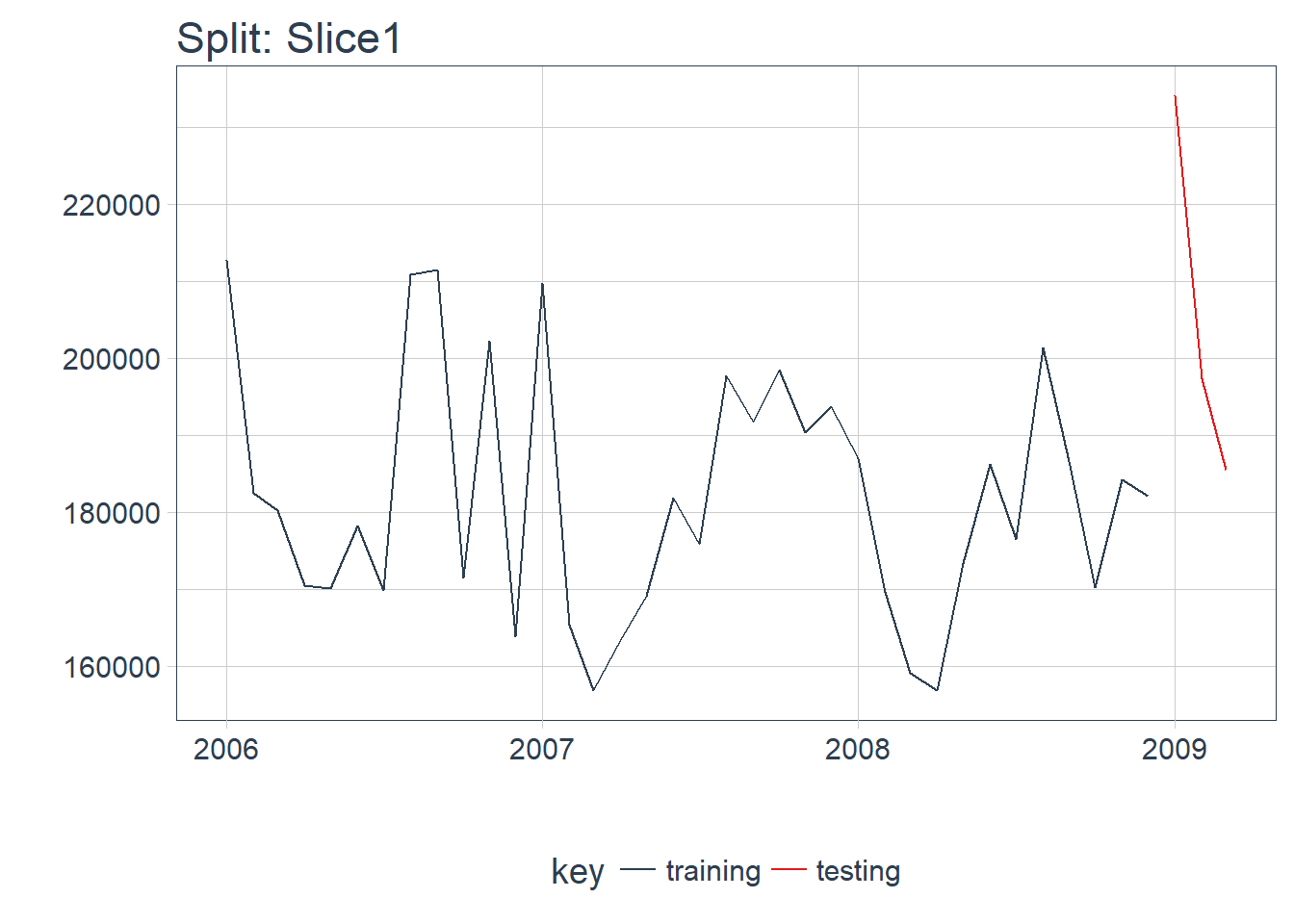Split test/train

df_trn <- training(split)
df_tst <- testing(split)

df <- bind_rows(
) %>%
as_tbl_time(index = index)

df
## # A time tibble: 39 x 3
## # Index: index
##    index        value key
##    <date>       <dbl> <chr>
##  1 2006-01-01 212711. training
##  2 2006-02-01 182493. training
##  3 2006-03-01 180162. training
##  4 2006-04-01 170443. training
##  5 2006-05-01 170079. training
##  6 2006-06-01 178162. training
##  7 2006-07-01 169873. training
##  8 2006-08-01 210880. training
##  9 2006-09-01 211548. training
## 10 2006-10-01 171406. training
## # ... with 29 more rows

Scale and center the data

We can process this data using the recipes package

rec_obj <- recipe(value ~ ., df) %>%
step_sqrt(value) %>%
step_center(value) %>%
step_scale(value) %>%
prep()

df_processed_tbl <- bake(rec_obj, df)

df_processed_tbl
## # A tibble: 39 x 3
##    index        value key
##    <date>       <dbl> <fct>
##  1 2006-01-01  1.62   training
##  2 2006-02-01 -0.0515 training
##  3 2006-03-01 -0.186  training
##  4 2006-04-01 -0.757  training
##  5 2006-05-01 -0.778  training
##  6 2006-06-01 -0.302  training
##  7 2006-07-01 -0.791  training
##  8 2006-08-01  1.52   training
##  9 2006-09-01  1.56   training
## 10 2006-10-01 -0.699  training
## # ... with 29 more rows

We will need to save the scaling factors so that we can restore the scale at the end

center_history <- rec_obj$steps[]$means["value"]
scale_history  <- rec_obj$steps[]$sds["value"]

c("center" = center_history, "scale" = scale_history)
## center.value  scale.value
##    428.24106     20.34574

Let’s define our model inputs:

# Model inputs
lag_setting  <- 3 # = nrow(df_tst)
batch_size   <- 3
train_length <- 24 # nrow(df_trn)
tsteps       <- 1
epochs       <- 300

Set up training and test sets

# Training Set
lag_train_tbl <- df_processed_tbl %>%
mutate(value_lag = lag(value, n = lag_setting)) %>%
filter(!is.na(value_lag)) %>%
filter(key == "training") %>%
tail(train_length)

x_train_vec <- lag_train_tbl$value_lag x_train_arr <- array(data = x_train_vec, dim = c(length(x_train_vec), 1, 1)) y_train_vec <- lag_train_tbl$value
y_train_arr <- array(data = y_train_vec, dim = c(length(y_train_vec), 1))

# Testing Set
lag_test_tbl <- df_processed_tbl %>%
mutate(
value_lag = lag(value, n = lag_setting)
) %>%
filter(!is.na(value_lag)) %>%
filter(key == "testing")

x_test_vec <- lag_test_tbl$value_lag x_test_arr <- array(data = x_test_vec, dim = c(length(x_test_vec), 1, 1)) y_test_vec <- lag_test_tbl$value
y_test_arr <- array(data = y_test_vec, dim = c(length(y_test_vec), 1))

Test a LSTM network to get a feel for the model:

model <- keras_model_sequential()

model %>%
layer_lstm(units            = 10,
input_shape      = c(tsteps, 1),
batch_size       = batch_size,
return_sequences = TRUE,
stateful         = TRUE) %>%
layer_lstm(units            = 10,
return_sequences = FALSE,
stateful         = TRUE) %>%
layer_dense(units = 1)

model %>%
compile(loss = 'mae', optimizer = 'adam')

model
## Model
## ___________________________________________________________________________
## Layer (type)                     Output Shape                  Param #
## ===========================================================================
## lstm_1 (LSTM)                    (3, 1, 10)                    480
## ___________________________________________________________________________
## lstm_2 (LSTM)                    (3, 10)                       840
## ___________________________________________________________________________
## dense_23 (Dense)                 (3, 1)                        11
## ===========================================================================
## Total params: 1,331
## Trainable params: 1,331
## Non-trainable params: 0
## ___________________________________________________________________________
for (i in 1:epochs) {
model %>% keras::fit(x   = x_train_arr,
y          = y_train_arr,
batch_size = batch_size,
epochs     = 1,
verbose    = 0,
shuffle    = FALSE)

model %>% reset_states()
# cat("Epoch: ", i, '\n')

}

Now let’s predict the next 3 months so we can test the performance of the model

pred_out <- model %>%
predict(x_test_arr, batch_size = batch_size) %>%
.[,1]

# Retransform values
pred_tbl <- tibble(
index   = lag_test_tbl$index, value = (pred_out * scale_history + center_history)^2 ) # Combine actual data with predictions tbl_1 <- df_trn %>% add_column(key = "actual") tbl_2 <- df_tst %>% add_column(key = "actual") tbl_3 <- pred_tbl %>% add_column(key = "predict") # Create time_bind_rows() to solve dplyr issue time_bind_rows <- function(data_1, data_2, index) { index_expr <- enquo(index) bind_rows(data_1, data_2) %>% as_tbl_time(index = !! index_expr) } ret <- list(tbl_1, tbl_2, tbl_3) %>% reduce(time_bind_rows, index = index) %>% arrange(key, index) %>% mutate(key = as_factor(key)) ret ## # A time tibble: 42 x 3 ## # Index: index ## index value key ## <date> <dbl> <fct> ## 1 2006-01-01 212711. actual ## 2 2006-02-01 182493. actual ## 3 2006-03-01 180162. actual ## 4 2006-04-01 170443. actual ## 5 2006-05-01 170079. actual ## 6 2006-06-01 178162. actual ## 7 2006-07-01 169873. actual ## 8 2006-08-01 210880. actual ## 9 2006-09-01 211548. actual ## 10 2006-10-01 171406. actual ## # ... with 32 more rows Function to calculate rmse for us calc_rmse <- function(prediction_tbl) { rmse_calculation <- function(data) { data %>% spread(key = key, value = value) %>% select(-index) %>% filter(!is.na(predict)) %>% rename( truth = actual, estimate = predict ) %>% rmse(truth, estimate) } safe_rmse <- possibly(rmse_calculation, otherwise = NA) safe_rmse(prediction_tbl) } which is: calc_rmse(ret) ##  32301.01 Function that plots the errors… # Setup single plot function plot_prediction <- function(data, id, alpha = 1, size = 2, base_size = 14) { rmse_val <- calc_rmse(data) g <- data %>% ggplot(aes(index, value, color = key)) + geom_point(alpha = alpha, size = size) + # geom_line(alpha = alpha, size = size)+ theme_tq(base_size = base_size) + scale_color_tq() + theme(legend.position = "none") + labs( title = glue("{id}, RMSE: {round(rmse_val, digits = 1)}"), x = "", y = "" ) return(g) } Let’s see the performance: ret %>% plot_prediction(id = split_id, alpha = 0.65) + theme(legend.position = "bottom")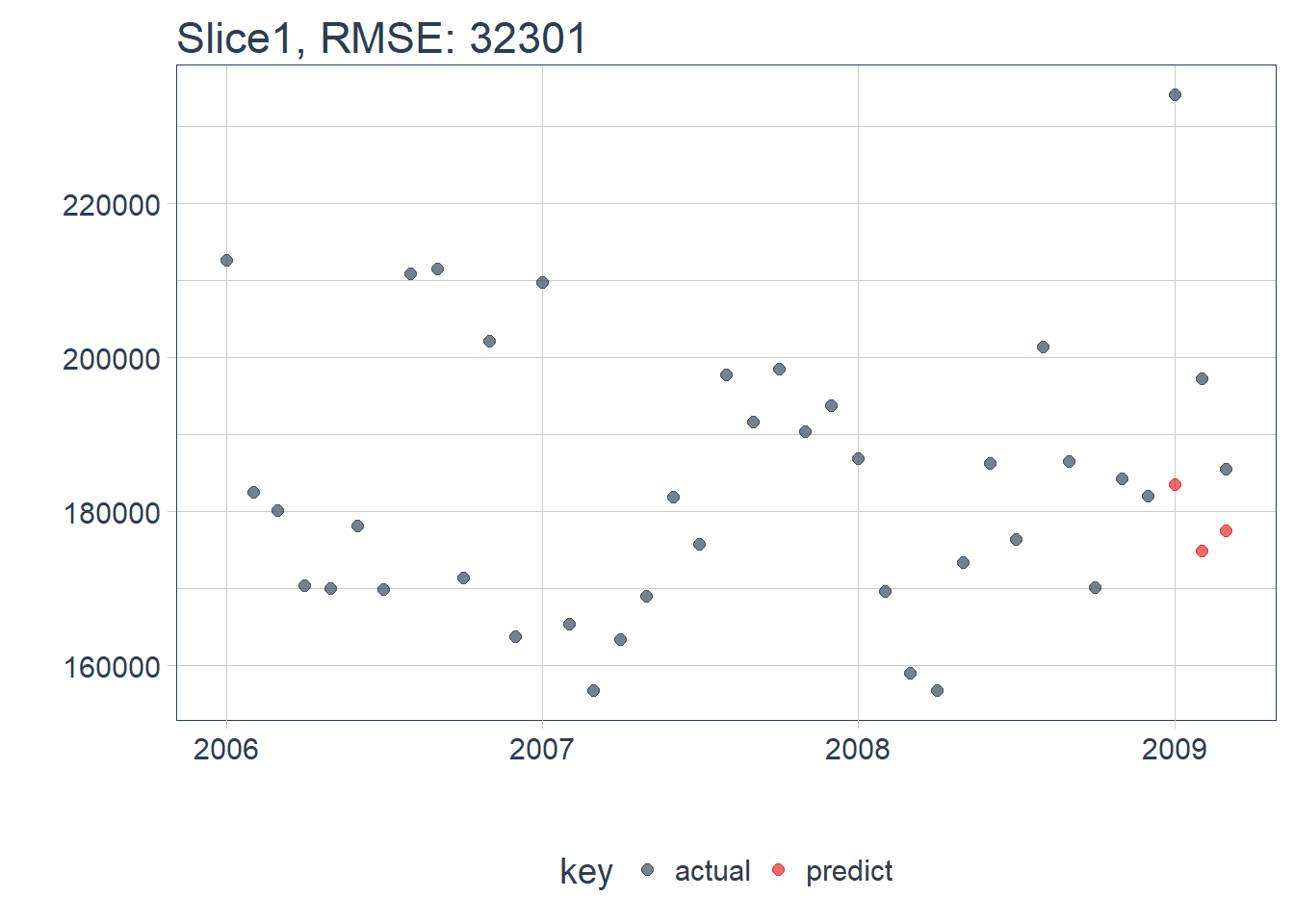Awesome the LSTM model is ready to start making us some predictions! Now we need to back test the model on all the time slices now since we now have a method we can map over all of them simultaneously Everything set… time to get started!Back test LSTM model Let’s create a function that will compile, fit and predict the LSTM network. Basically this function will do everything we just did for testing the single fold so we can map it accross all the folds predict_keras_lstm <- function(split, epochs = 300, lag_setting, batch_size, train_length, tsteps, optimizer = 'adam',...) { lstm_prediction <- function(split, epochs, lag_setting, batch_size, train_length, tsteps, optimizer, ...) { # 5.1.2 Data Setup df_trn <- training(split) df_tst <- testing(split) df <- bind_rows( df_trn %>% add_column(key = "training"), df_tst %>% add_column(key = "testing") ) %>% as_tbl_time(index = index) # 5.1.3 Preprocessing rec_obj <- recipe(value ~ ., df) %>% step_sqrt(value) %>% step_center(value) %>% step_scale(value) %>% prep() df_processed_tbl <- bake(rec_obj, df) center_history <- rec_obj$steps[]$means["value"] scale_history <- rec_obj$steps[]$sds["value"] # # 5.1.4 LSTM Plan # lag_setting <- 120 # = nrow(df_tst) # batch_size <- 40 # train_length <- 440 # tsteps <- 1 # epochs <- epochs # 5.1.5 Train/Test Setup lag_train_tbl <- df_processed_tbl %>% mutate(value_lag = lag(value, n = lag_setting)) %>% filter(!is.na(value_lag)) %>% filter(key == "training") %>% tail(train_length) x_train_vec <- lag_train_tbl$value_lag
x_train_arr <- array(data = x_train_vec, dim = c(length(x_train_vec), 1, 1))

y_train_vec <- lag_train_tbl$value y_train_arr <- array(data = y_train_vec, dim = c(length(y_train_vec), 1)) lag_test_tbl <- df_processed_tbl %>% mutate( value_lag = lag(value, n = lag_setting) ) %>% filter(!is.na(value_lag)) %>% filter(key == "testing") x_test_vec <- lag_test_tbl$value_lag
x_test_arr <- array(data = x_test_vec, dim = c(length(x_test_vec), 1, 1))

y_test_vec <- lag_test_tbl$value y_test_arr <- array(data = y_test_vec, dim = c(length(y_test_vec), 1)) # 5.1.6 LSTM Model model <- keras_model_sequential() model %>% layer_lstm(units = 10, input_shape = c(tsteps, 1), batch_size = batch_size, return_sequences = TRUE, stateful = TRUE) %>% layer_lstm(units = 10, return_sequences = FALSE, stateful = TRUE) %>% layer_dense(units = 1) model %>% compile(loss = 'mae', optimizer = optimizer) # 5.1.7 Fitting LSTM for (i in 1:epochs) { model %>% fit(x = x_train_arr, y = y_train_arr, batch_size = batch_size, epochs = 1, verbose = 0, shuffle = FALSE) model %>% reset_states() # cat("Epoch: ", i, '\n') } # 5.1.8 Predict and Return Tidy Data # Make Predictions pred_out <- model %>% predict(x_test_arr, batch_size = batch_size) %>% .[,1] # Retransform values pred_tbl <- tibble( index = lag_test_tbl$index,
value   = (pred_out * scale_history + center_history)^2
)

# Combine actual data with predictions
tbl_1 <- df_trn %>%

tbl_2 <- df_tst %>%

tbl_3 <- pred_tbl %>%

# Create time_bind_rows() to solve dplyr issue
time_bind_rows <- function(data_1, data_2, index) {
index_expr <- enquo(index)
bind_rows(data_1, data_2) %>%
as_tbl_time(index = !! index_expr)
}

ret <- list(tbl_1, tbl_2, tbl_3) %>%
reduce(time_bind_rows, index = index) %>%
arrange(key, index) %>%
mutate(key = as_factor(key))

return(ret)

}

safe_lstm <- possibly(lstm_prediction, otherwise = NA)

safe_lstm(split, epochs, lag_setting, batch_size, train_length,  tsteps, optimizer, ...)

}

Set our test parameters (remember that the training samples must be divisable by the batch size)

lag_setting  <- 3 # = nrow(df_tst)
batch_size   <- 3
train_length <- 24 # nrow(df_trn)
tsteps       <- 1
epochs       <- 300

Now we map the LSTM model over all our times series folds to back test:

sample_predictions_lstm_tbl <-
rolling_origin_resamples %>%
mutate(predict = map(
splits,
predict_keras_lstm,
epochs = 300,
lag_setting = lag_setting,
batch_size = batch_size,
train_length = train_length ,
tsteps = tsteps ,
))

The same way we created a funtion to plot the test and training sets we can make one to plot the actuals and predicted values:

plot_predictions <- function(sampling_tbl, predictions_col,
ncol = 3, alpha = 1, size = 2, base_size = 14,
title = "Backtested Predictions") {

predictions_col_expr <- enquo(predictions_col)

# Map plot_split() to sampling_tbl
sampling_tbl_with_plots <- sampling_tbl %>%
mutate(gg_plots = map2(!! predictions_col_expr, id,
.f        = plot_prediction,
alpha     = alpha,
size      = size,
base_size = base_size))

# Make plots with cowplot
plot_list <- sampling_tbl_with_plots$gg_plots p_temp <- plot_list[] + theme(legend.position = "bottom") legend <- get_legend(p_temp) p_body <- plot_grid(plotlist = plot_list, ncol = ncol) p_title <- ggdraw() + draw_label(title, size = 18, fontface = "bold", colour = palette_light()[]) g <- plot_grid(p_title, p_body, legend, ncol = 1, rel_heights = c(0.05, 1, 0.05)) return(g) } Let’s see how our LSTM model did: sample_predictions_lstm_tbl %>% plot_predictions(predictions_col = predict, alpha = 0.5, size = 1, base_size = 10, title = "Keras Stateful LSTM: Backtested Predictions")Seems to be working! Even though we had small correlations We can reuse our rmse calculator to see our accuracy over each time slice: sample_rmse_tbl <- sample_predictions_lstm_tbl %>% mutate(rmse = map_dbl(predict, calc_rmse)) %>% select(id, rmse) sample_rmse_tbl ## # Rolling origin forecast resampling ## # A tibble: 3 x 2 ## id rmse ## * <chr> <dbl> ## 1 Slice1 37492. ## 2 Slice2 20870. ## 3 Slice3 8719. We can plot the RMSE over the various folds: sample_rmse_tbl %>% ggplot(aes(rmse)) + geom_histogram(aes(y = ..density..), fill = palette_light()[], bins = 5) + geom_density(fill = palette_light()[], alpha = 0.5) + theme_tq() + ggtitle("Histogram of RMSE")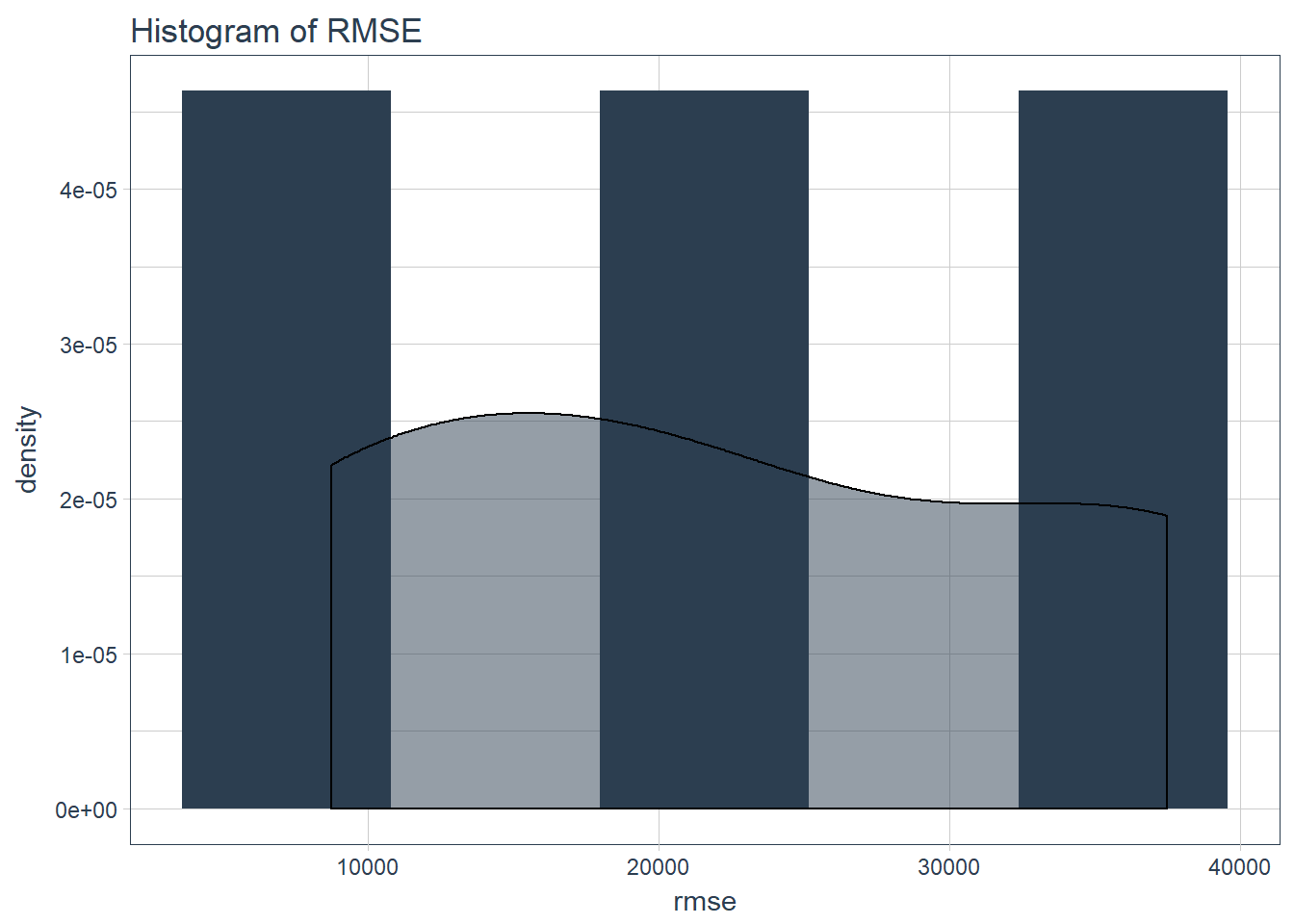And the distribution is: sample_rmse_tbl %>% summarize( mean_rmse = mean(rmse), sd_rmse = sd(rmse) ) ## # Rolling origin forecast resampling ## # A tibble: 1 x 2 ## mean_rmse sd_rmse ## <dbl> <dbl> ## 1 22360. 14444. Test final model on full dataset Now we create our function that will test the LSTM model on all the data: predict_keras_lstm_future <- function(data, epochs = 300,lag_setting, batch_size, train_length, tsteps, optimizer = 'adam',...) { lstm_prediction <- function(data, epochs,lag_setting, batch_size, train_length, tsteps, optimizer, ...) { # 5.1.2 Data Setup (MODIFIED) df <- data # 5.1.3 Preprocessing rec_obj <- recipe(value ~ ., df) %>% step_sqrt(value) %>% step_center(value) %>% step_scale(value) %>% prep() df_processed_tbl <- bake(rec_obj, df) center_history <- rec_obj$steps[]$means["value"] scale_history <- rec_obj$steps[]$sds["value"] # 5.1.5 Train Setup (MODIFIED) lag_train_tbl <- df_processed_tbl %>% mutate(value_lag = lag(value, n = lag_setting)) %>% filter(!is.na(value_lag)) %>% tail(train_length) x_train_vec <- lag_train_tbl$value_lag
x_train_arr <- array(data = x_train_vec, dim = c(length(x_train_vec), 1, 1))

y_train_vec <- lag_train_tbl$value y_train_arr <- array(data = y_train_vec, dim = c(length(y_train_vec), 1)) x_test_vec <- y_train_vec %>% tail(lag_setting) x_test_arr <- array(data = x_test_vec, dim = c(length(x_test_vec), 1, 1)) # 5.1.6 LSTM Model model <- keras_model_sequential() model %>% layer_lstm(units = 10, input_shape = c(tsteps, 1), batch_size = batch_size, return_sequences = TRUE, stateful = TRUE) %>% layer_lstm(units = 10, return_sequences = FALSE, stateful = TRUE) %>% layer_dense(units = 1) model %>% compile(loss = 'mae', optimizer = optimizer) # 5.1.7 Fitting LSTM for (i in 1:epochs) { model %>% fit(x = x_train_arr, y = y_train_arr, batch_size = batch_size, epochs = 1, verbose = 0, shuffle = FALSE) model %>% reset_states() # cat("Epoch: ", i, '\n') } # 5.1.8 Predict and Return Tidy Data (MODIFIED) # Make Predictions pred_out <- model %>% predict(x_test_arr, batch_size = batch_size) %>% .[,1] # Make future index using tk_make_future_timeseries() idx <- data %>% tk_index() %>% tk_make_future_timeseries(n_future = lag_setting) # Retransform values pred_tbl <- tibble( index = idx, value = (pred_out * scale_history + center_history)^2 ) # Combine actual data with predictions tbl_1 <- df %>% add_column(key = "actual") tbl_3 <- pred_tbl %>% add_column(key = "predict") # Create time_bind_rows() to solve dplyr issue time_bind_rows <- function(data_1, data_2, index) { index_expr <- enquo(index) bind_rows(data_1, data_2) %>% as_tbl_time(index = !! index_expr) } ret <- list(tbl_1, tbl_3) %>% reduce(time_bind_rows, index = index) %>% arrange(key, index) %>% mutate(key = as_factor(key)) return(ret) } safe_lstm <- possibly(lstm_prediction, otherwise = NA) safe_lstm(data, epochs, lag_setting, batch_size, train_length, tsteps, optimizer, ...) } future_LSTM_tbl <- predict_keras_lstm_future(LSTM_train, epochs = 300, lag_setting = lag_setting, batch_size = batch_size, train_length = train_length , tsteps = tsteps , optimizer = 'adam' ) future_LSTM_tbl %>% # filter_time("2008" ~ "end") %>% plot_prediction(id = NULL, alpha = 0.4, size = 1.5) + theme(legend.position = "bottom") + ggtitle("House prices: 3 Month Forecast using LSTM deep neural net", subtitle = "Using the full dataset")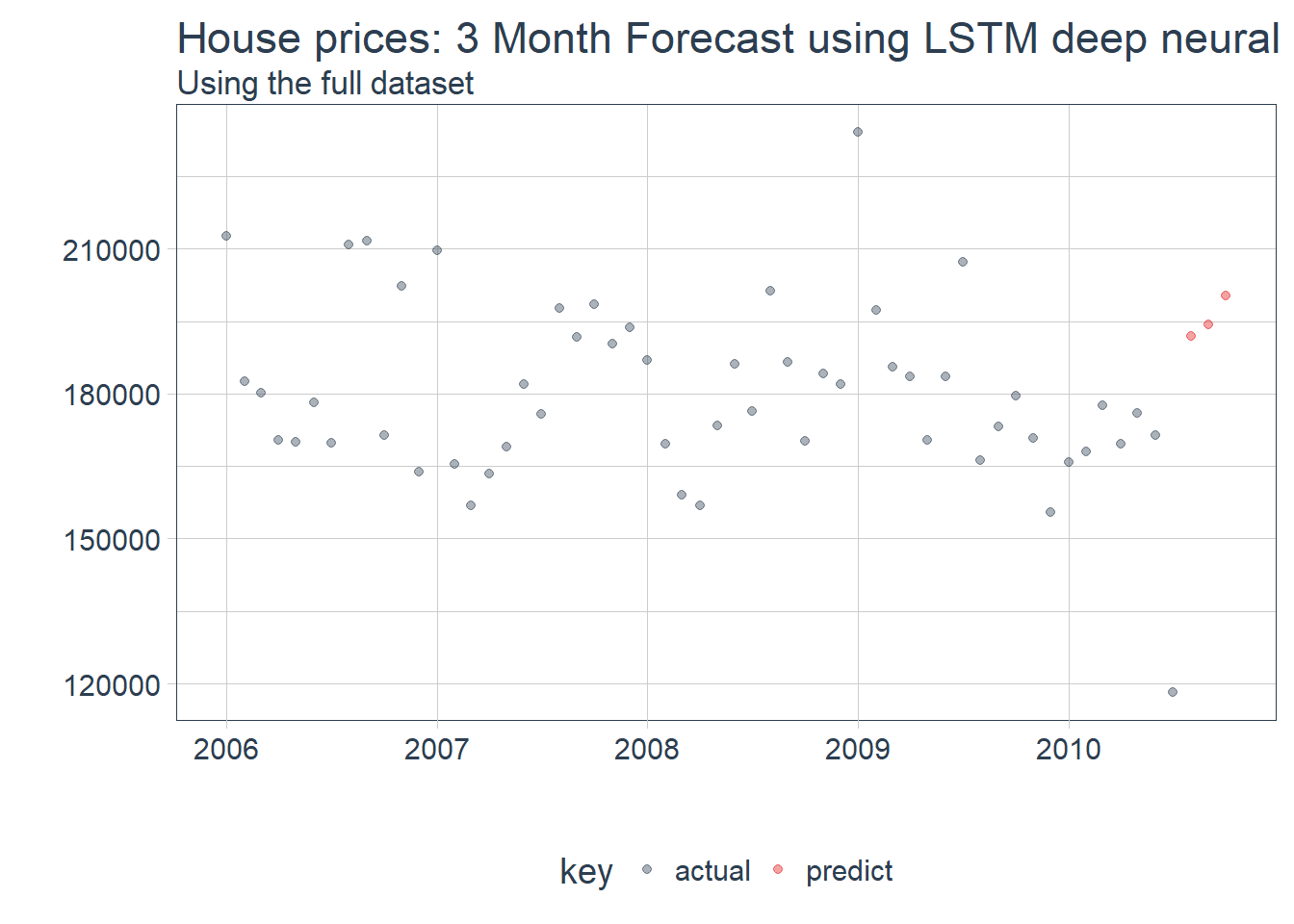Combine LSTM and Inference networks into 1 deep neural network?? I was tempted to combine these 2 networks together so that I could backpropegate them simultaneously. This would allow us to use the inference network using descriptive features together with the macro economic trends in the market of housing prices. To stack different deep neural networks together we can use the keras API like this: Define complete model # data.frame(factor = 1:100,res = 2324/1:100) %>% filter(res == trunc(res)) # batch_size <- 28 batch_size <- 83 inference_input <- layer_input( name = "inference_input",batch_shape = c(batch_size,265)) encoded_inference_network <- inference_input %>% layer_dense(units = 128, activation = 'relu') %>% # 1 axis and nr features = number columns layer_batch_normalization() %>% # layer_dropout(rate = 0.2) %>% layer_dense(units = 64, activation ='relu') %>% layer_dense(units = 32, activation ='relu') # layer_dense(units = 25, activation ='relu') %>% LSTM_input <- layer_input(name = "LSTM_input",batch_shape = c(batch_size,1,1)) encoded_LSTM_network <- LSTM_input %>% layer_lstm(units = 100, batch_size = batch_size, return_sequences = TRUE, stateful = TRUE) %>% layer_lstm(units = 100, return_sequences = FALSE, stateful = TRUE) concatenated <- layer_concatenate(list(encoded_LSTM_network, encoded_inference_network)) house_price <- concatenated %>% layer_dense(units = 5, activation = "relu") %>% layer_dense(units = 1) model <- keras_model(list(LSTM_input, inference_input), house_price) model %>% compile( optimizer = "adam", loss='mse', metrics = c('mae') ) Create our LSTM input: I realised very quickly that we have a small problem here… To backpropogate both networks simultaneously they would need to feed the same batch of inputs into both networks! But our LSTM model was trained on monthly data - this means I had a 1 to many relationship between the 2 models inputs. I tried to naively circumvent this by infering the lag of prices on a per house level. We could do this by simply using the average shift and applying it as a predicted lag index: prepare_LSTM_input <- function(monthly_ts,sample_ts,lag_setting,train_length) { # 5.1.5 Train Setup (MODIFIED) lag_train_tbl <- monthly_ts %>% mutate(value_lag_month = lag(value, n = lag_setting)) %>% filter(!is.na(value_lag_month)) %>% tail(train_length) %>% rename(value_month = value) join_df <- sample_ts %>% left_join(lag_train_tbl, by = 'index') %>% mutate(value_lag = value * value_lag_month/value_month) %>% select(index,value,value_lag) rec_obj <- recipe(index ~ ., join_df) %>% step_sqrt(all_predictors()) %>% step_center(all_predictors()) %>% step_scale(all_predictors()) %>% prep() df_processed_tbl <- bake(rec_obj, join_df) center_history <- rec_obj$steps[]$means["value"] scale_history <- rec_obj$steps[]$sds["value"] x_train_vec <- df_processed_tbl$value_lag
x_train_arr <- array(data = x_train_vec, dim = c(length(x_train_vec), 1, 1))

y_train_vec <- df_processed_tbl$value y_train_arr <- array(data = y_train_vec, dim = c(length(y_train_vec), 1)) return(list(LSTM_input = x_train_arr, LSTM_target = y_train_arr, center_history = center_history, scale_history = scale_history)) } return_original_scale <- function(pred_out, scale_history,center_history) { return((pred_out * scale_history + center_history)^2) } sample_ts <- train %>% select(SalePrice,Yr.Sold,Mo.Sold) %>% tidyr::unite(col = index,Yr.Sold,Mo.Sold,sep="-") %>% mutate(index = index %>% zoo::as.yearmon() %>% as_date()) %>% rename(value = SalePrice) %>% as_tbl_time(index = index) LSTM_input_full_model <- prepare_LSTM_input(monthly_ts = LSTM_train,sample_ts = sample_ts,lag_setting = 3,train_length = 24) So let’s try to train the 2 deep neural networks simulteneously: history <- model %>% fit( list(inference_input = inference_train_X, LSTM_input = LSTM_input_full_model$LSTM_input),
LSTM_input_full_model\$LSTM_target,
epochs = 300,
batch_size = batch_size,
shuffle = FALSE
,verbose = 0
)

The network can’t back propogate properly! This is because the LSTM model needs the inputs to move consistently through time. Even though our inputs are correctly lagged we have inconsistent numbers of rows for each time index which ruins the ‘memory’ part of the model…

What we can do however is use the prediction of price movement as a feature in the inference model. We can train the inference model by adding the future average price of properties by month by region truncating that data that has no future observations.

Then we train the LSTM and inference networks seperately - the LSTM then predicts that future movement for us so we can test the accuracy of our combined model feeding the LSTM into the inference network into a result